# Homework 1: COVID-19 Cases Prediction (Regression)

## Simple Baseline

### Some Utilities

You do not need to modify this part.

### Preprocess

We have three kinds of datasets:

• train: for training
• dev: for validation
• test: for testing (w/o target value)

#### Dataset

The COVID19Dataset below does:

• read .csv files
• extract features
• split covid.train.csv into train/dev sets
• normalize features

Finishing TODO below might make you pass medium baseline.

A DataLoader loads data from a given Dataset into batches.

### Deep Neural Network

NeuralNet is an nn.Module designed for regression.
The DNN consists of 2 fully-connected layers with ReLU activation.
This module also included a function cal_loss for calculating loss.

### Setup Hyper-parameters

config contains hyper-parameters for training and the path to save your model.

### Start Training!

Saving model (epoch =    1, loss =  78.8524)
Saving model (epoch =    2, loss =  37.6170)
Saving model (epoch =    3, loss =  26.1203)
Saving model (epoch =    4, loss =  16.1862)
Saving model (epoch =    5, loss =  9.7153)
Saving model (epoch =    6, loss =  6.3701)
Saving model (epoch =    7, loss =  5.1802)
Saving model (epoch =    8, loss =  4.4255)
Saving model (epoch =    9, loss =  3.8009)
Saving model (epoch =   10, loss =  3.3691)
Saving model (epoch =   11, loss =  3.0943)
Saving model (epoch =   12, loss =  2.8176)
Saving model (epoch =   13, loss =  2.6274)
Saving model (epoch =   14, loss =  2.4542)
Saving model (epoch =   15, loss =  2.3012)
Saving model (epoch =   16, loss =  2.1766)
Saving model (epoch =   17, loss =  2.0641)
Saving model (epoch =   18, loss =  1.9399)
Saving model (epoch =   19, loss =  1.8978)
Saving model (epoch =   20, loss =  1.7950)
Saving model (epoch =   21, loss =  1.7164)
Saving model (epoch =   22, loss =  1.6455)
Saving model (epoch =   23, loss =  1.5912)
Saving model (epoch =   24, loss =  1.5599)
Saving model (epoch =   25, loss =  1.5197)
Saving model (epoch =   26, loss =  1.4698)
Saving model (epoch =   27, loss =  1.4189)
Saving model (epoch =   28, loss =  1.3992)
Saving model (epoch =   29, loss =  1.3696)
Saving model (epoch =   30, loss =  1.3442)
Saving model (epoch =   31, loss =  1.3231)
Saving model (epoch =   32, loss =  1.2834)
Saving model (epoch =   33, loss =  1.2804)
Saving model (epoch =   34, loss =  1.2471)
Saving model (epoch =   36, loss =  1.2414)
Saving model (epoch =   37, loss =  1.2138)
Saving model (epoch =   38, loss =  1.2083)
Saving model (epoch =   41, loss =  1.1591)
Saving model (epoch =   42, loss =  1.1484)
Saving model (epoch =   44, loss =  1.1209)
Saving model (epoch =   47, loss =  1.1122)
Saving model (epoch =   48, loss =  1.0937)
Saving model (epoch =   50, loss =  1.0842)
Saving model (epoch =   53, loss =  1.0655)
Saving model (epoch =   54, loss =  1.0613)
Saving model (epoch =   57, loss =  1.0524)
Saving model (epoch =   58, loss =  1.0394)
Saving model (epoch =   60, loss =  1.0267)
Saving model (epoch =   63, loss =  1.0248)
Saving model (epoch =   66, loss =  1.0099)
Saving model (epoch =   70, loss =  0.9829)
Saving model (epoch =   72, loss =  0.9817)
Saving model (epoch =   73, loss =  0.9743)
Saving model (epoch =   75, loss =  0.9671)
Saving model (epoch =   78, loss =  0.9643)
Saving model (epoch =   79, loss =  0.9597)
Saving model (epoch =   85, loss =  0.9549)
Saving model (epoch =   86, loss =  0.9535)
Saving model (epoch =   90, loss =  0.9467)
Saving model (epoch =   92, loss =  0.9432)
Saving model (epoch =   93, loss =  0.9231)
Saving model (epoch =   95, loss =  0.9127)
Saving model (epoch =  104, loss =  0.9117)
Saving model (epoch =  107, loss =  0.8994)
Saving model (epoch =  110, loss =  0.8935)
Saving model (epoch =  116, loss =  0.8882)
Saving model (epoch =  124, loss =  0.8872)
Saving model (epoch =  128, loss =  0.8724)
Saving model (epoch =  134, loss =  0.8722)
Saving model (epoch =  139, loss =  0.8677)
Saving model (epoch =  146, loss =  0.8654)
Saving model (epoch =  156, loss =  0.8642)
Saving model (epoch =  159, loss =  0.8528)
Saving model (epoch =  167, loss =  0.8494)
Saving model (epoch =  173, loss =  0.8492)
Saving model (epoch =  176, loss =  0.8461)
Saving model (epoch =  178, loss =  0.8403)
Saving model (epoch =  182, loss =  0.8375)
Saving model (epoch =  199, loss =  0.8295)
Saving model (epoch =  212, loss =  0.8273)
Saving model (epoch =  235, loss =  0.8252)
Saving model (epoch =  238, loss =  0.8233)
Saving model (epoch =  251, loss =  0.8211)
Saving model (epoch =  253, loss =  0.8205)
Saving model (epoch =  258, loss =  0.8175)
Saving model (epoch =  284, loss =  0.8143)
Saving model (epoch =  308, loss =  0.8136)
Saving model (epoch =  312, loss =  0.8075)
Saving model (epoch =  324, loss =  0.8045)
Saving model (epoch =  400, loss =  0.8040)
Saving model (epoch =  404, loss =  0.8010)
Saving model (epoch =  466, loss =  0.7998)
Saving model (epoch =  525, loss =  0.7993)
Saving model (epoch =  561, loss =  0.7945)
Saving model (epoch =  584, loss =  0.7903)
Saving model (epoch =  667, loss =  0.7896)
Saving model (epoch =  717, loss =  0.7823)
Saving model (epoch =  776, loss =  0.7812)
Saving model (epoch =  835, loss =  0.7797)
Saving model (epoch =  866, loss =  0.7771)
Saving model (epoch =  919, loss =  0.7770)
Saving model (epoch =  933, loss =  0.7748)
Saving model (epoch =  965, loss =  0.7705)
Saving model (epoch =  1027, loss =  0.7674)
Saving model (epoch =  1119, loss =  0.7647)
Saving model (epoch =  1140, loss =  0.7643)
Saving model (epoch =  1196, loss =  0.7620)
Saving model (epoch =  1234, loss =  0.7616)
Saving model (epoch =  1243, loss =  0.7582)
Finished training after 1444 epochs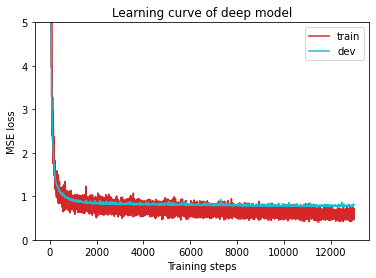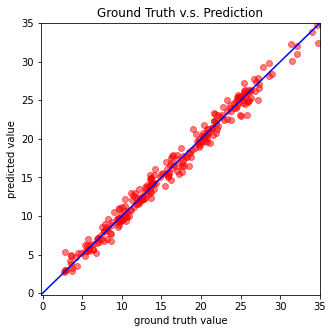### Testing

The predictions of your model on testing set will be stored at pred.csv.

Saving results to pred.csv


## Medium Baseline

Simple Baseline Code中标注了一些TODO，TA说只要完成这些TODO也许就可以达到Medium Baseline，TODO一共有这些：

1. TODO: Using 40 states & 2 tested_positive features (indices = 57 & 75)
2. TODO: How to modify this model to achieve better performance?
3. TODO: you may implement L1/L2 regularization here
4. TODO: How to tune these hyper-parameters to improve your model’s performance?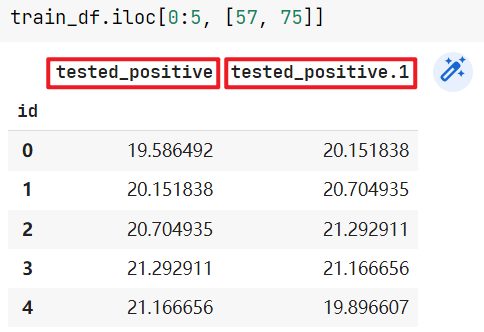## Strong Baseline

TA给了以下提示

1. Feature selection (what other features are useful?)
2. DNN architecture (layers? dimension? activation function?)
3. Training (mini-batch? optimizer? learning rate?)
4. L2 regularization
5. There are some mistakes in the sample code, can you find them?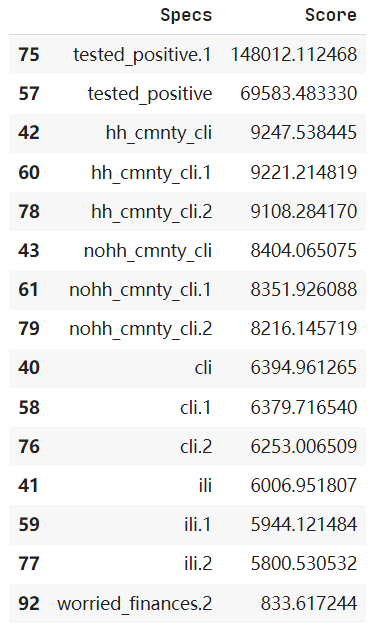TA说使用L2正则化，但其实效果提升不大

L1正则化的损失函数

L2正则化的损失函数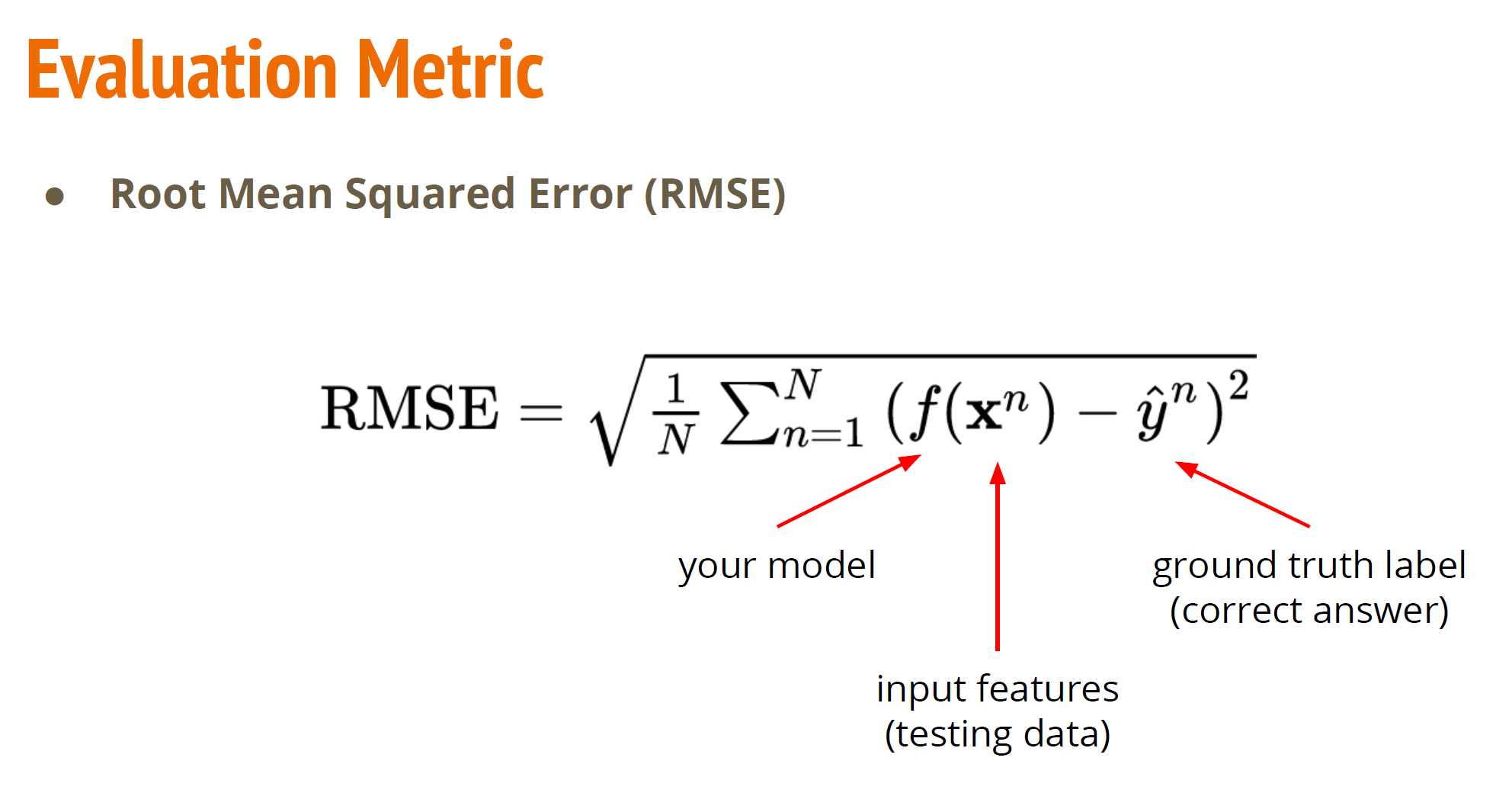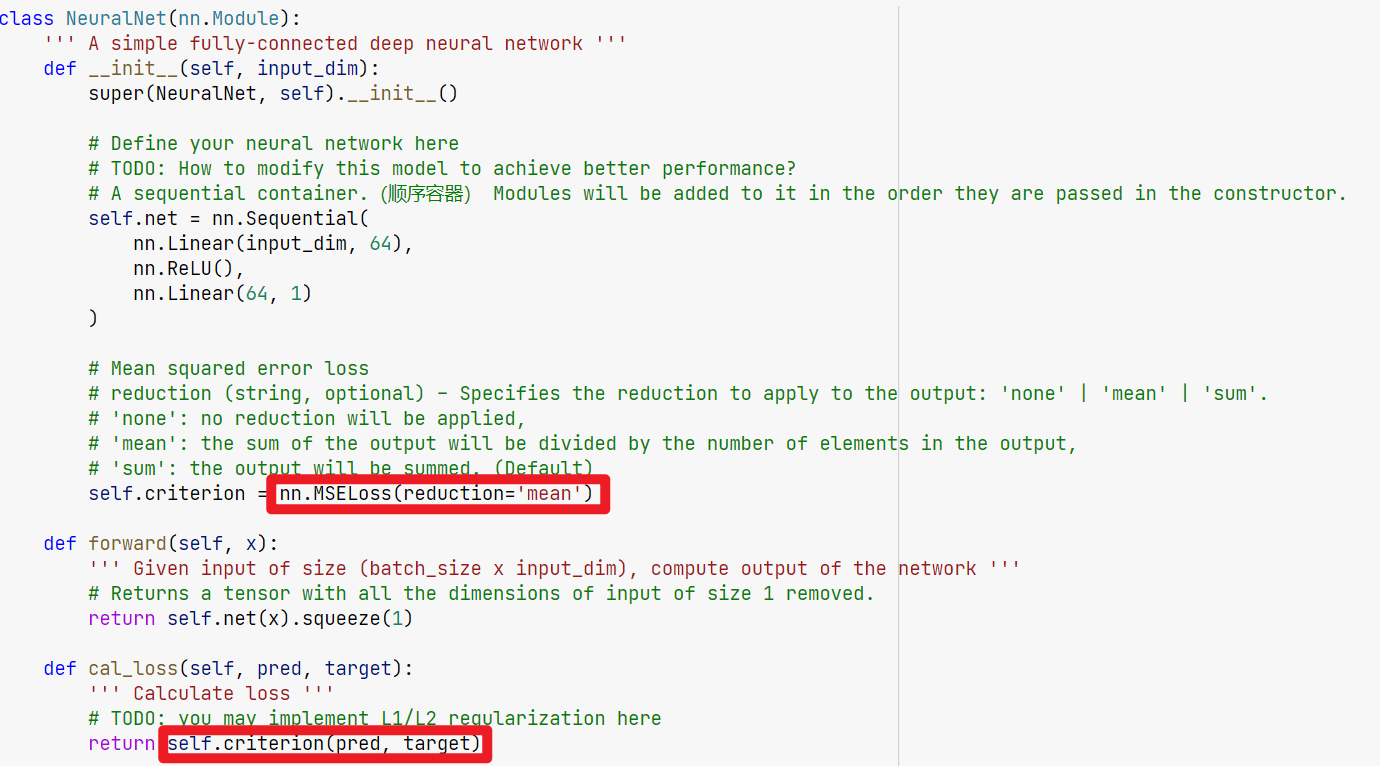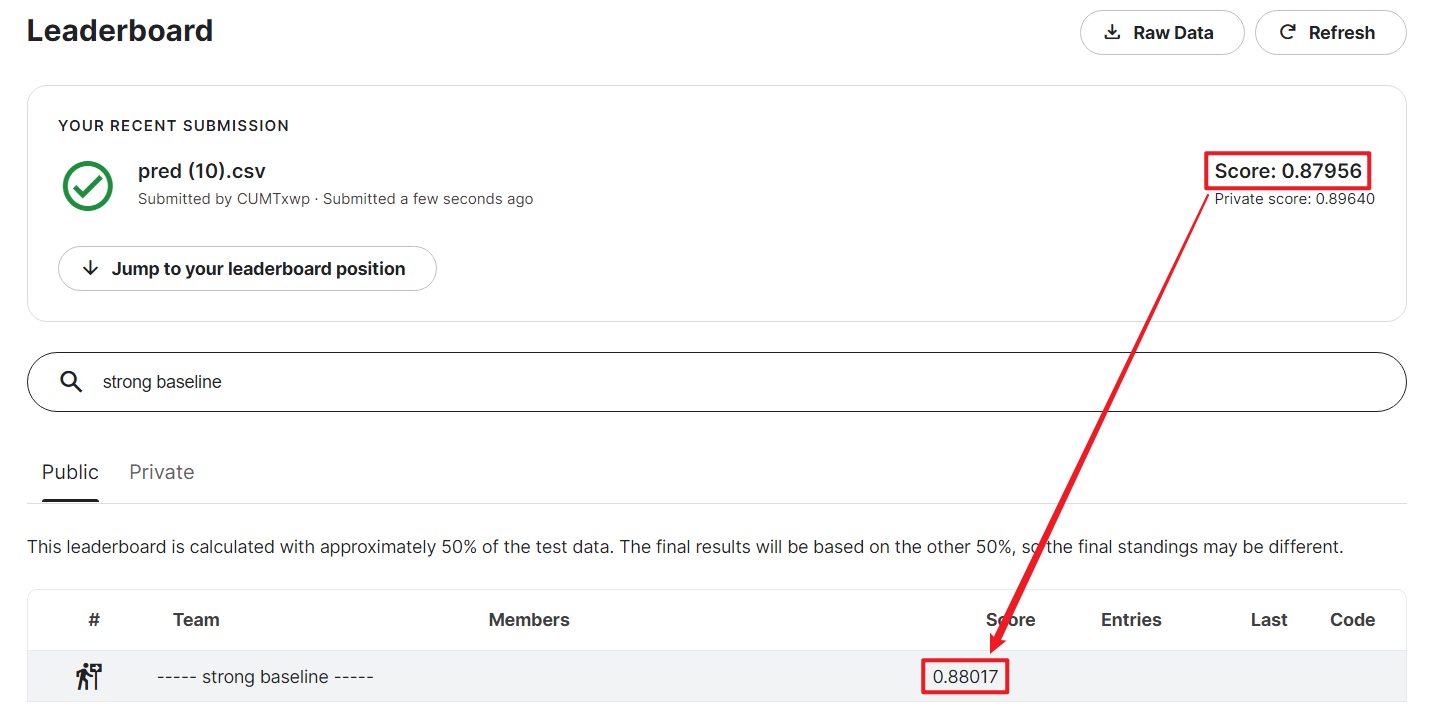# Homework 2: TIMIT framewise phoneme (classification)

## Phoneme Classification - Simple Baseline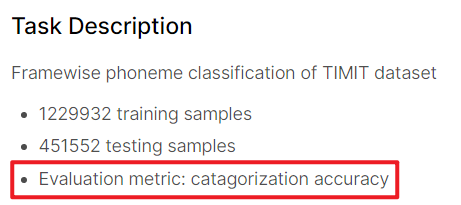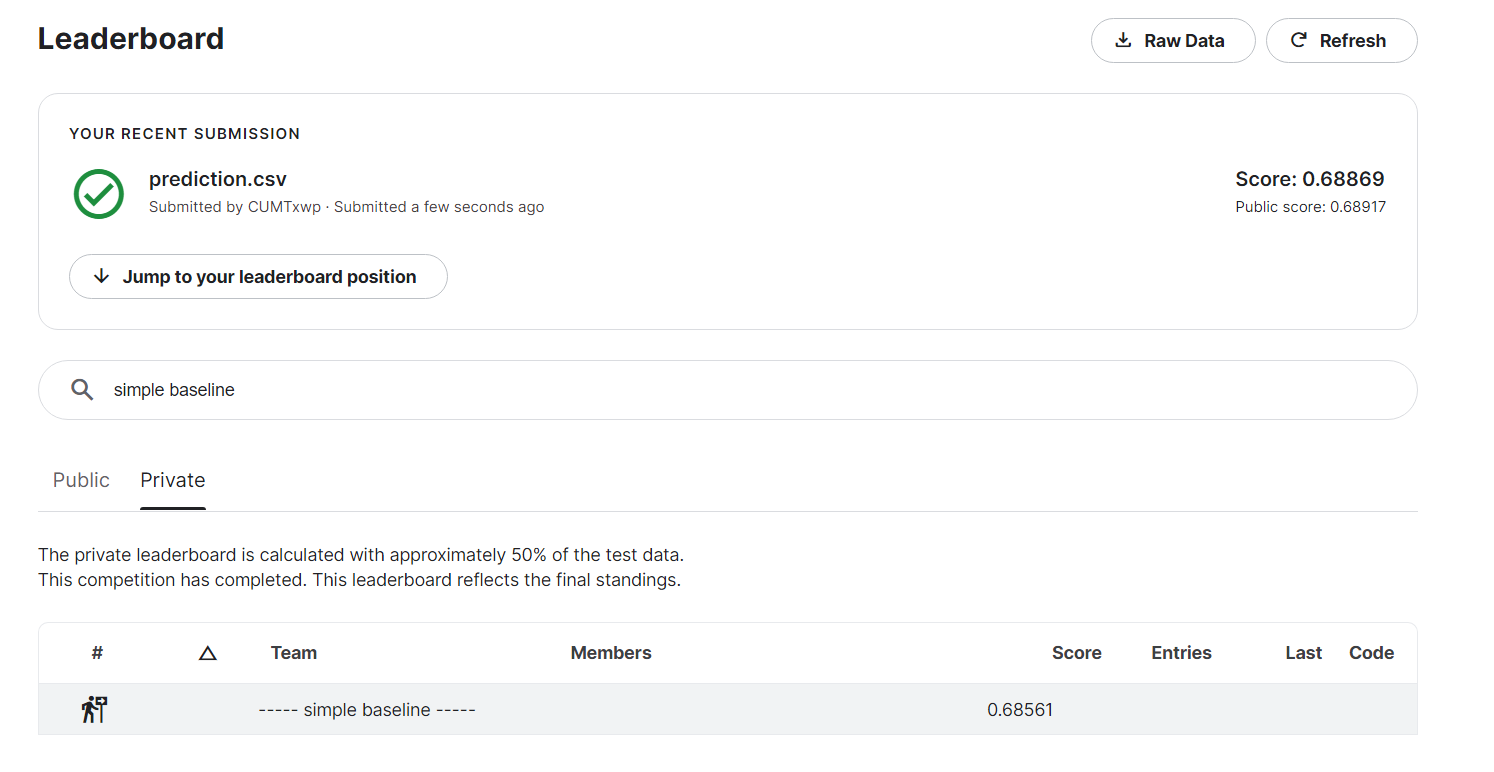ps：跑一次挺慢的，colab挂上GPU也要15min左右一次。

CODE:

## Phoneme Classification - Strong Baseline

TA在PPT里给了一些Hint。

1. Model architecture (layers? dimension? activation function?)
2. Training (batch size? optimizer? learning rate? epoch?)
3. Tips (batch norm? dropout? regularization?)

1-百万级数据集，所以VAL_RATIO设为5%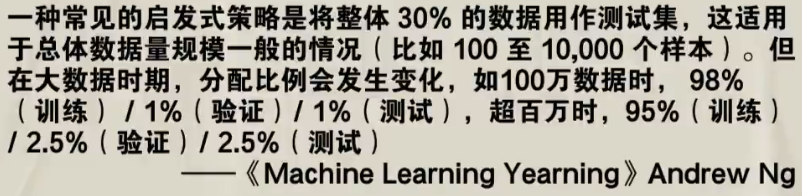# Homework 3: Convolutional Neural Network - Image Classfication(CNN)

## Simple BaselineTA’s Slide: Build a convolutional neural network using labeled images with provided codes.

## Medium Baseline

TA’s Slide: Improve the performance using labeled images with different model architectures or data augmentations.

TA给了两个方向different model architectures或者data augmentations。

（好像model architectures要使用resnet？）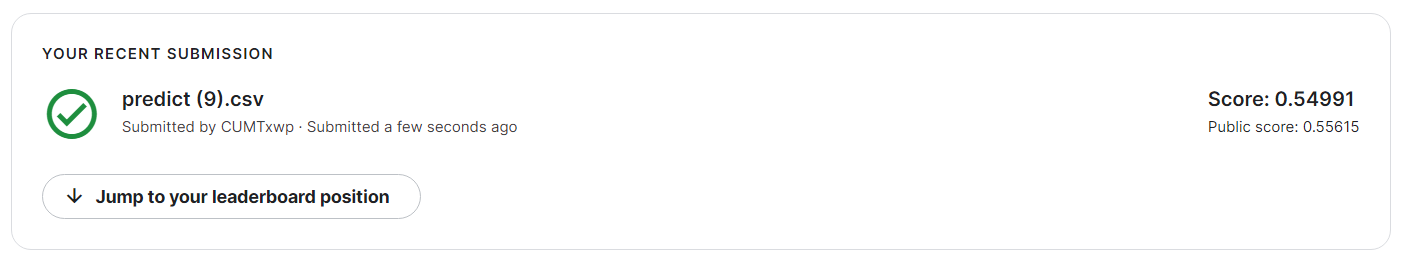## Strong Baseline

TA’s Slide: Improve the performance with additional unlabeled images.

TA将本次作业数据集中的大部分labeled images变成了unlabeled images，这里也是想要让学生练习Semi-supervised Learning（自监督学习）。

1. 当验证集最好精度达到一定值时再开始semi-supervised learning，否则model对unlabel datas的label不准确。
2. 为了避免semi-supervised learning影响效率，每semi_turns轮进行一次pseudo。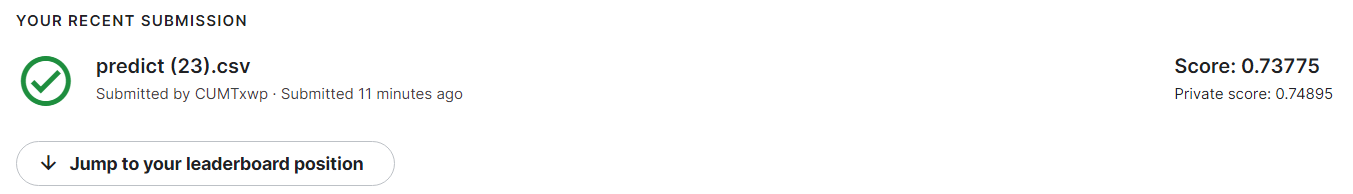# Course中的小知识点

## 如何降低loss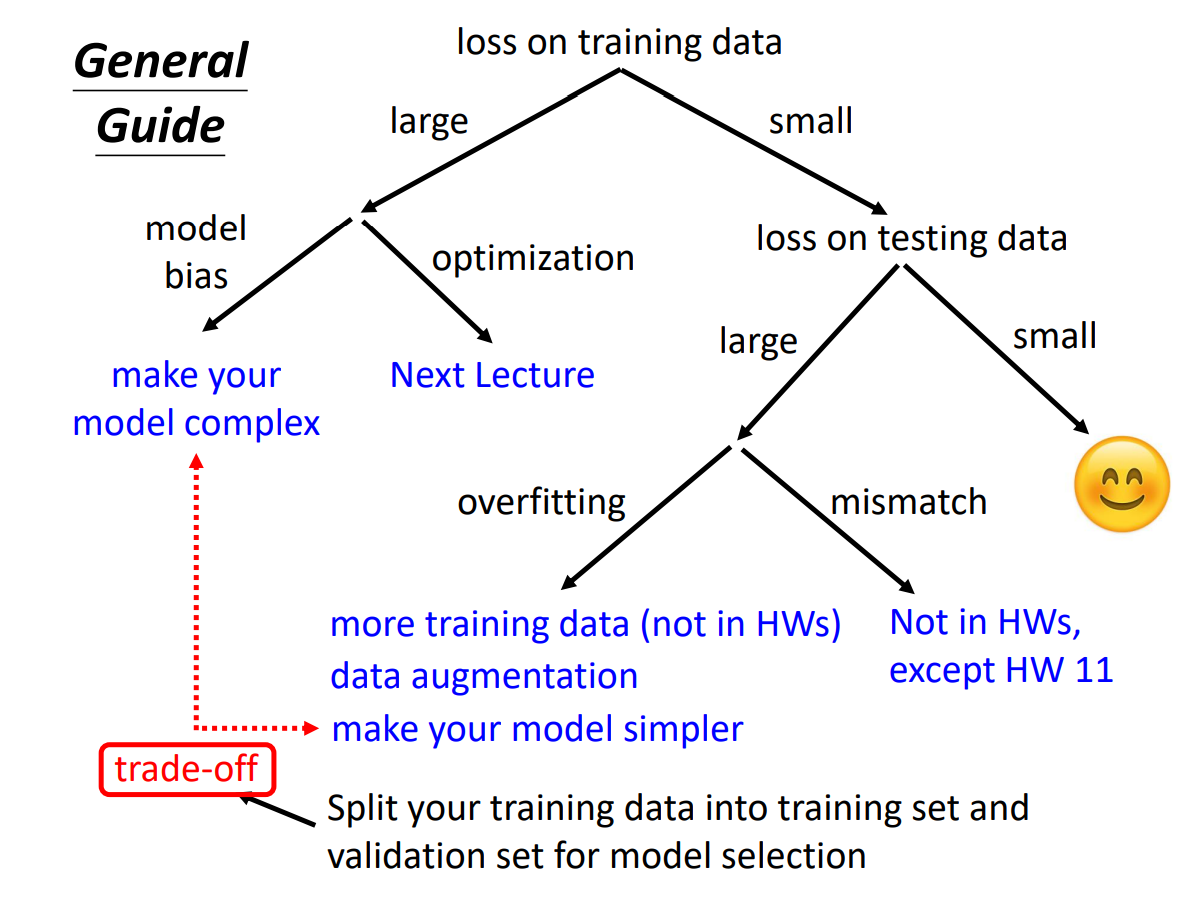## data Augmentation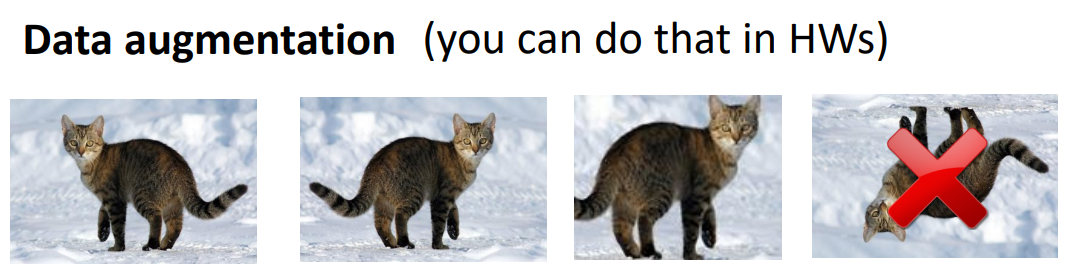## cross validation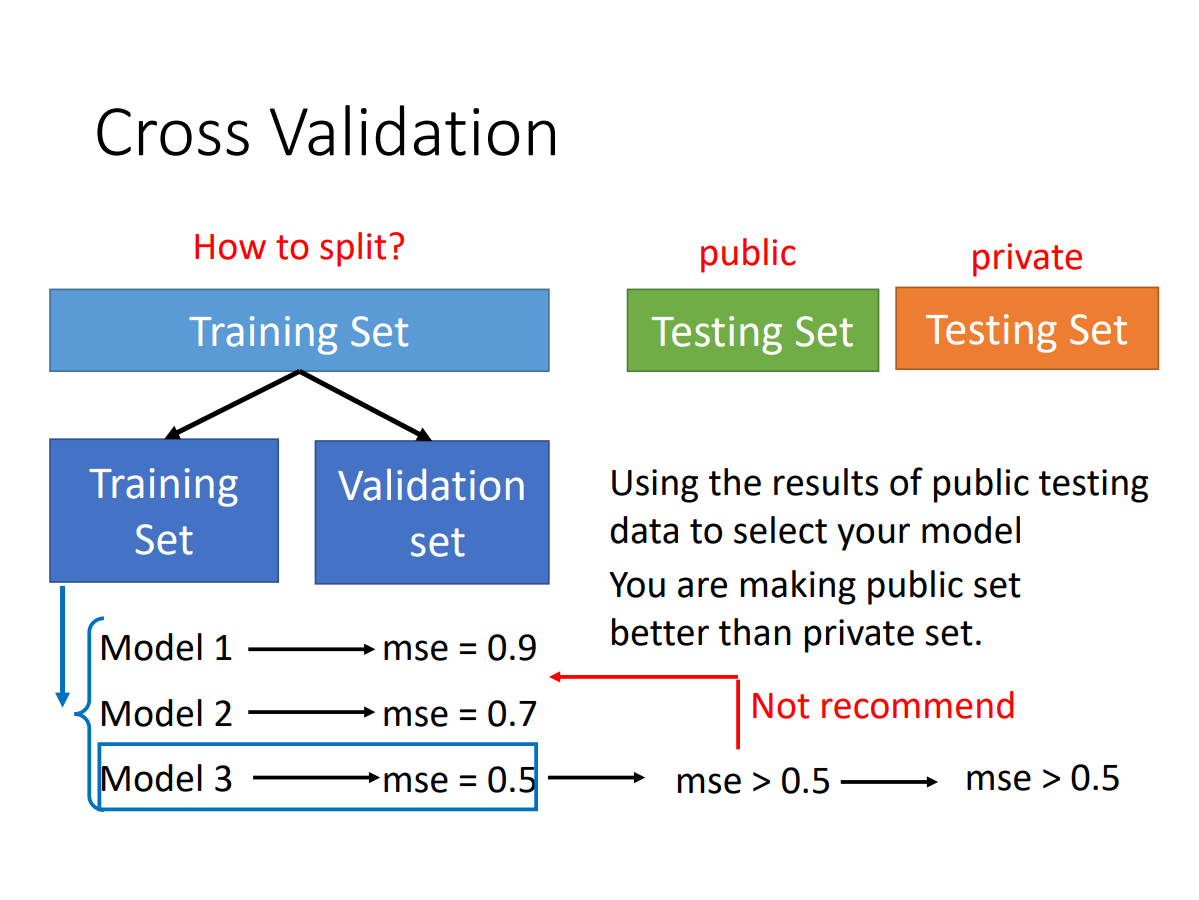## k-fold cross validation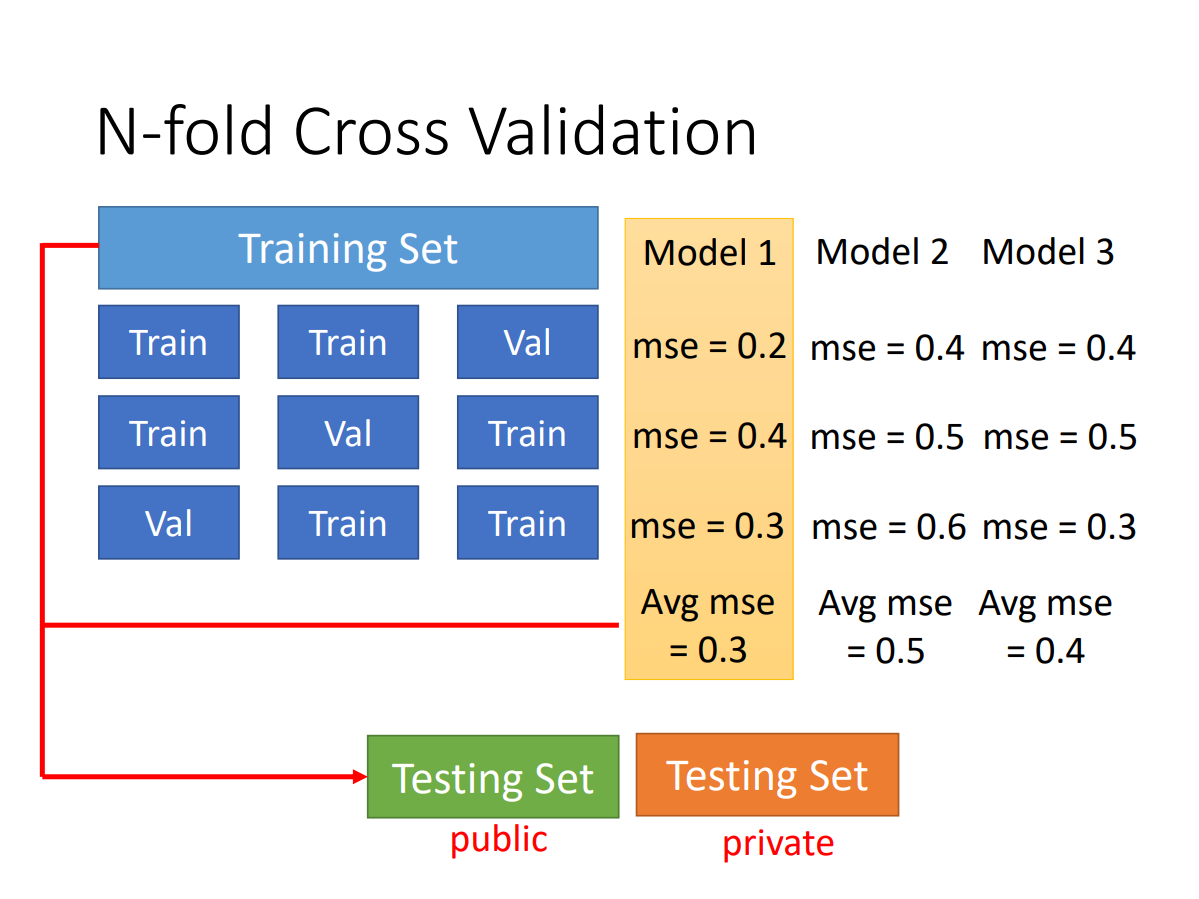## overfitting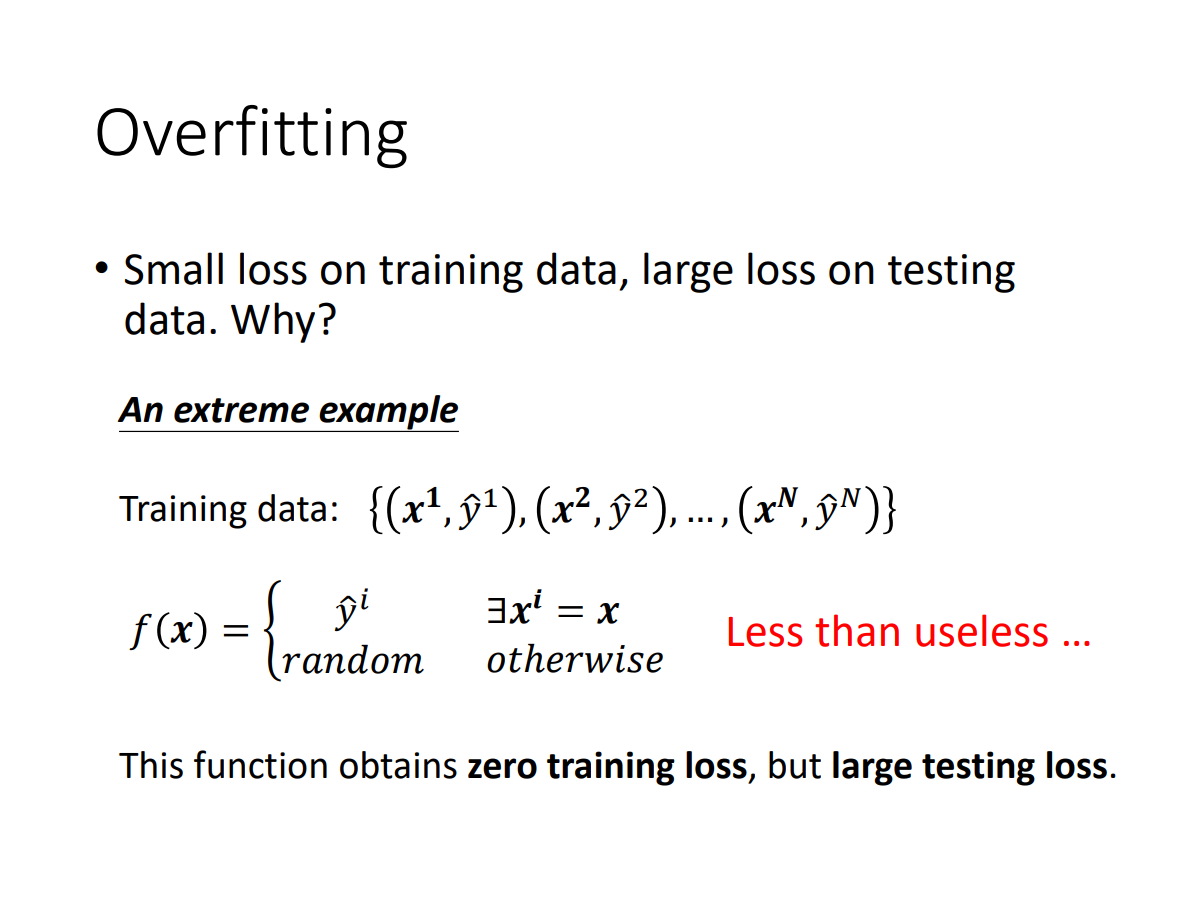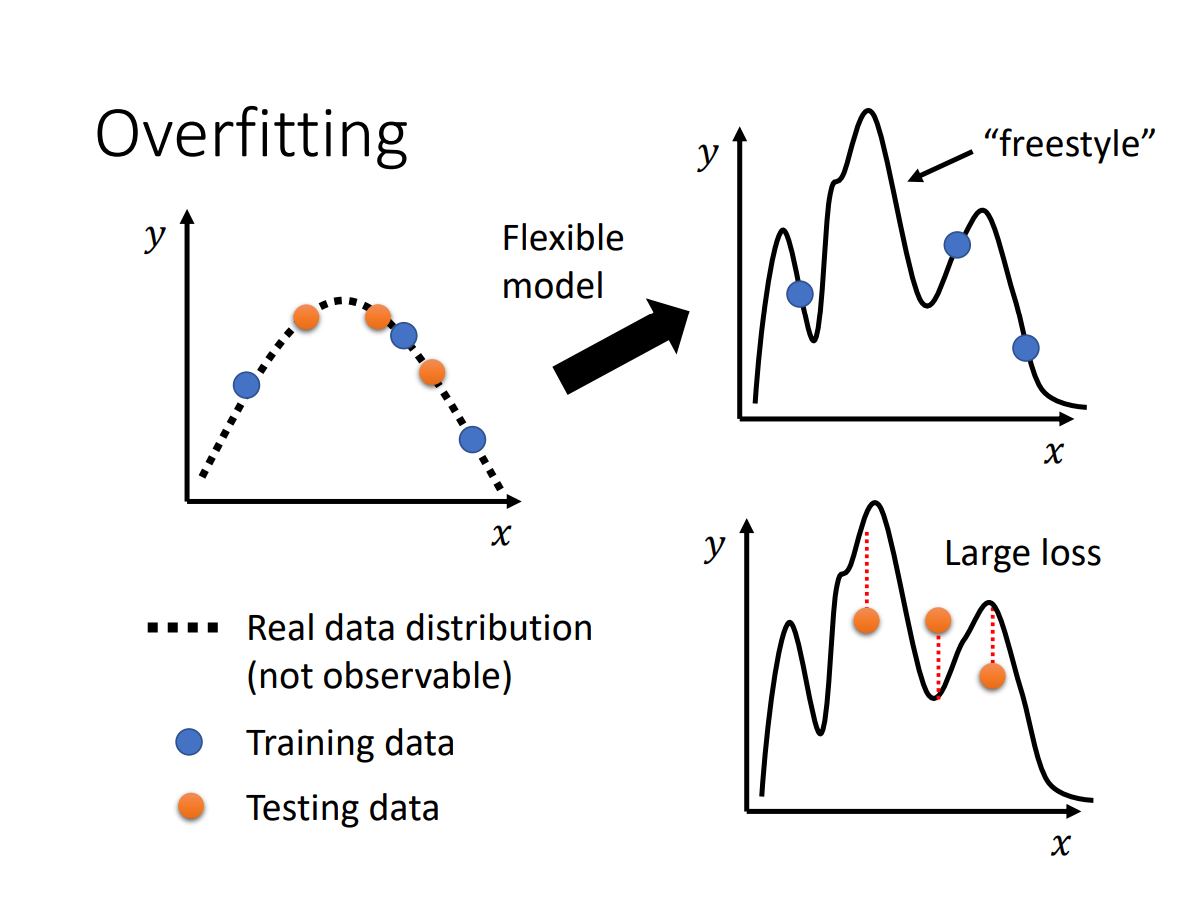model的flexibility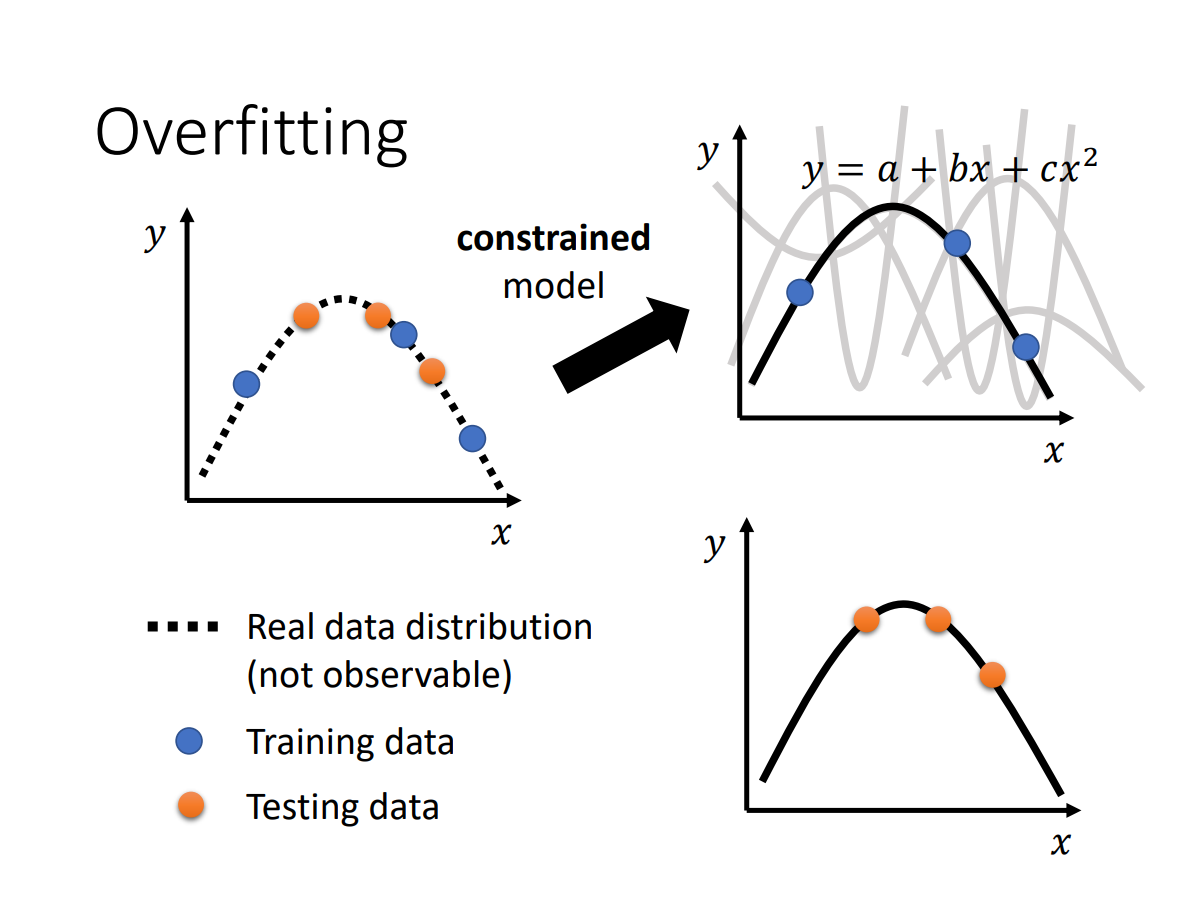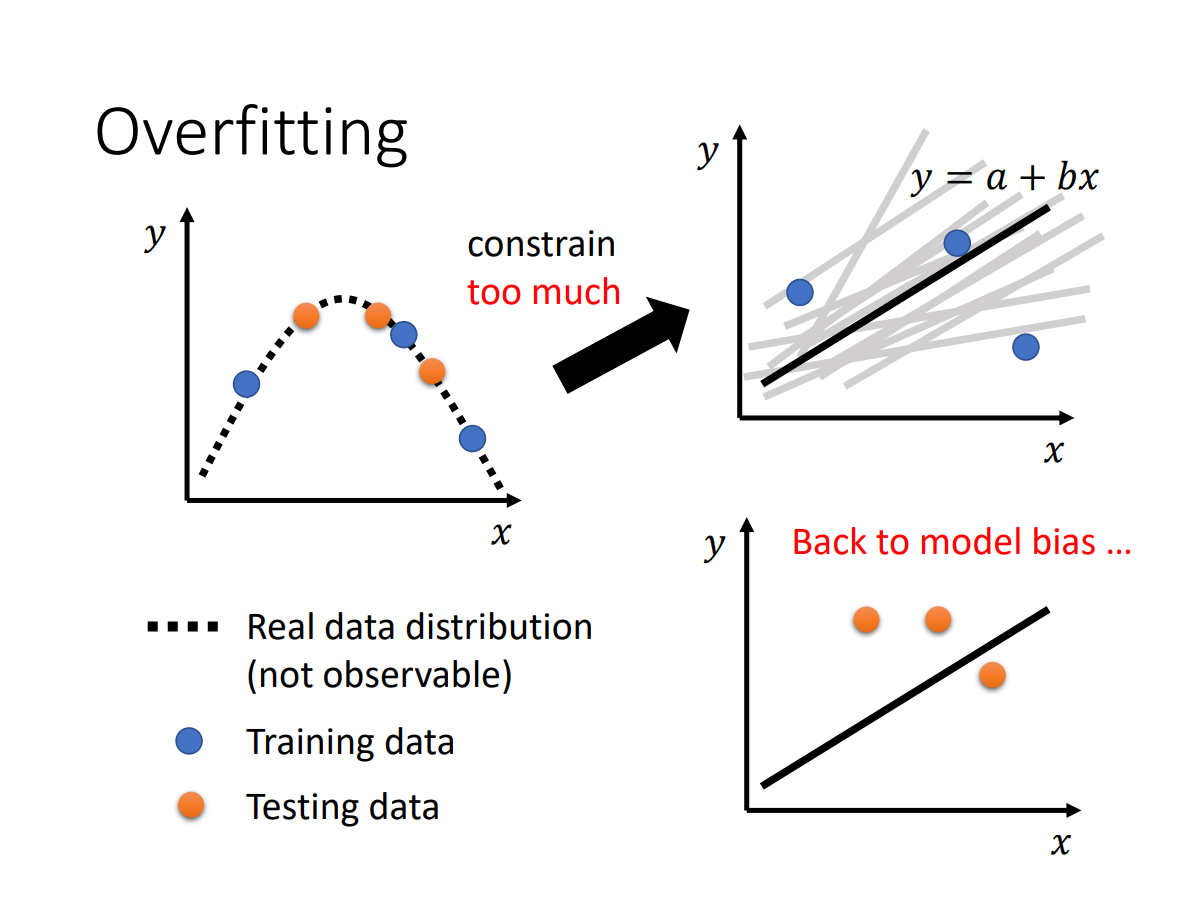## mismatch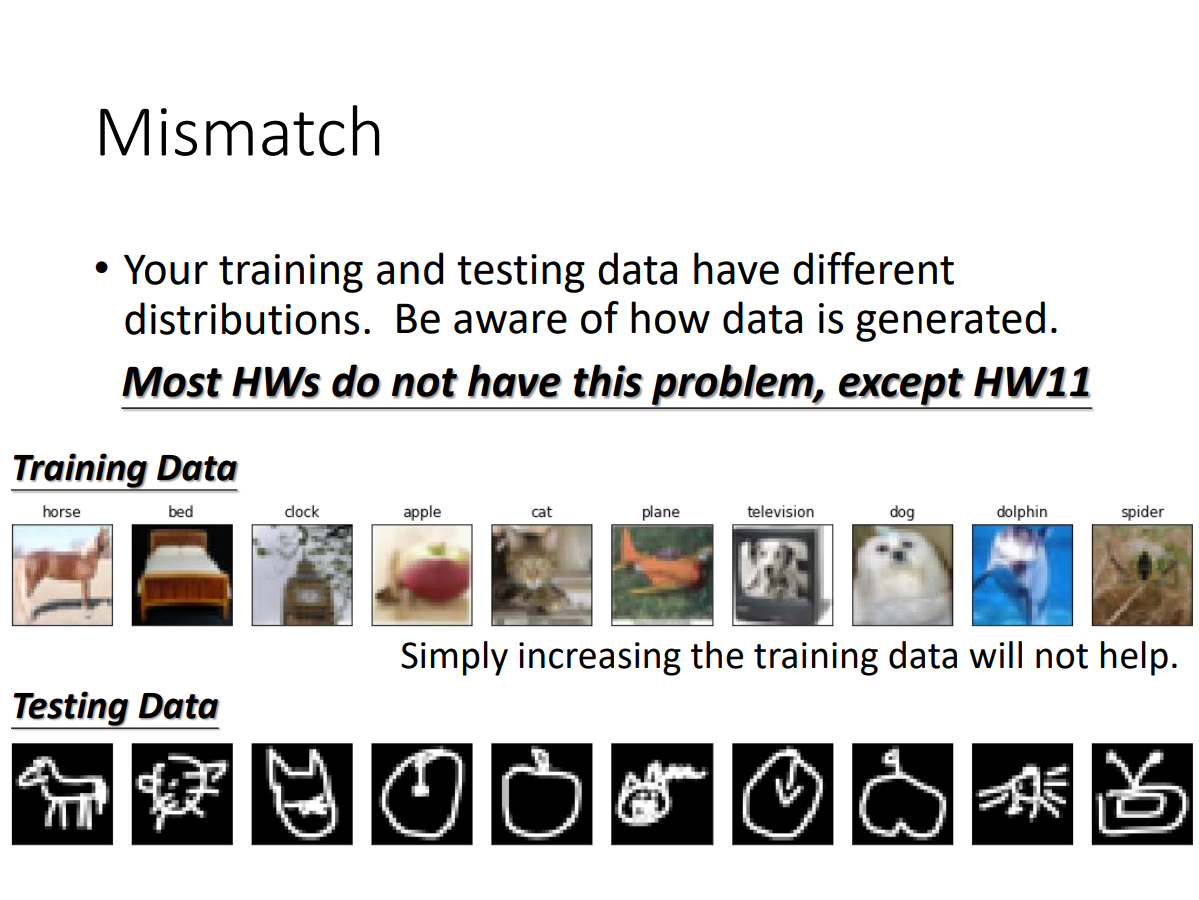eg.

Hung-yi Lee：感谢大家为了让这个模型不准，上周五花了很多力气去点了这个video，所以这一天（2021.2.26）是今年观看人数最多的一天！

（哈哈哈哈笑死我了）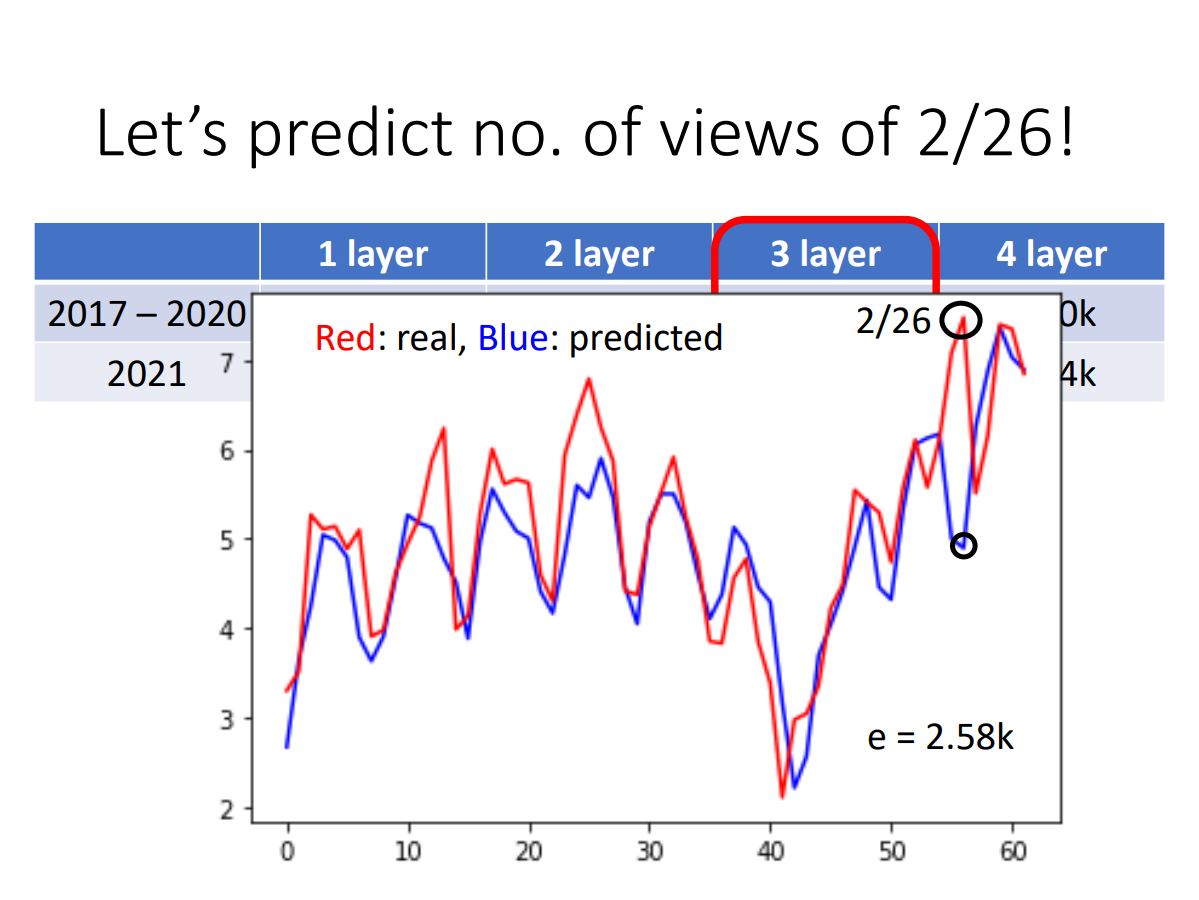## flat minima/sharp minima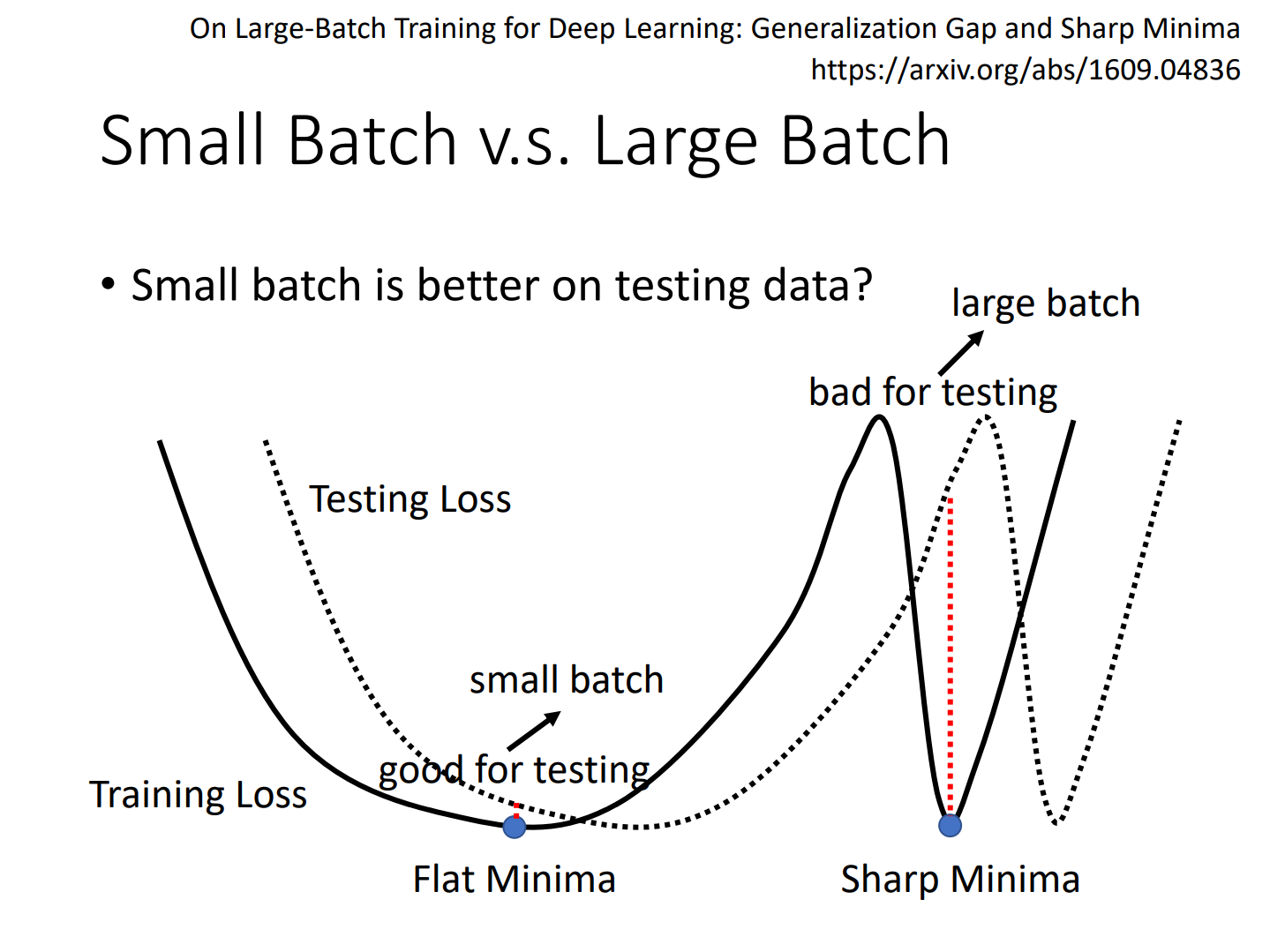## vanilla GD/GD with momentum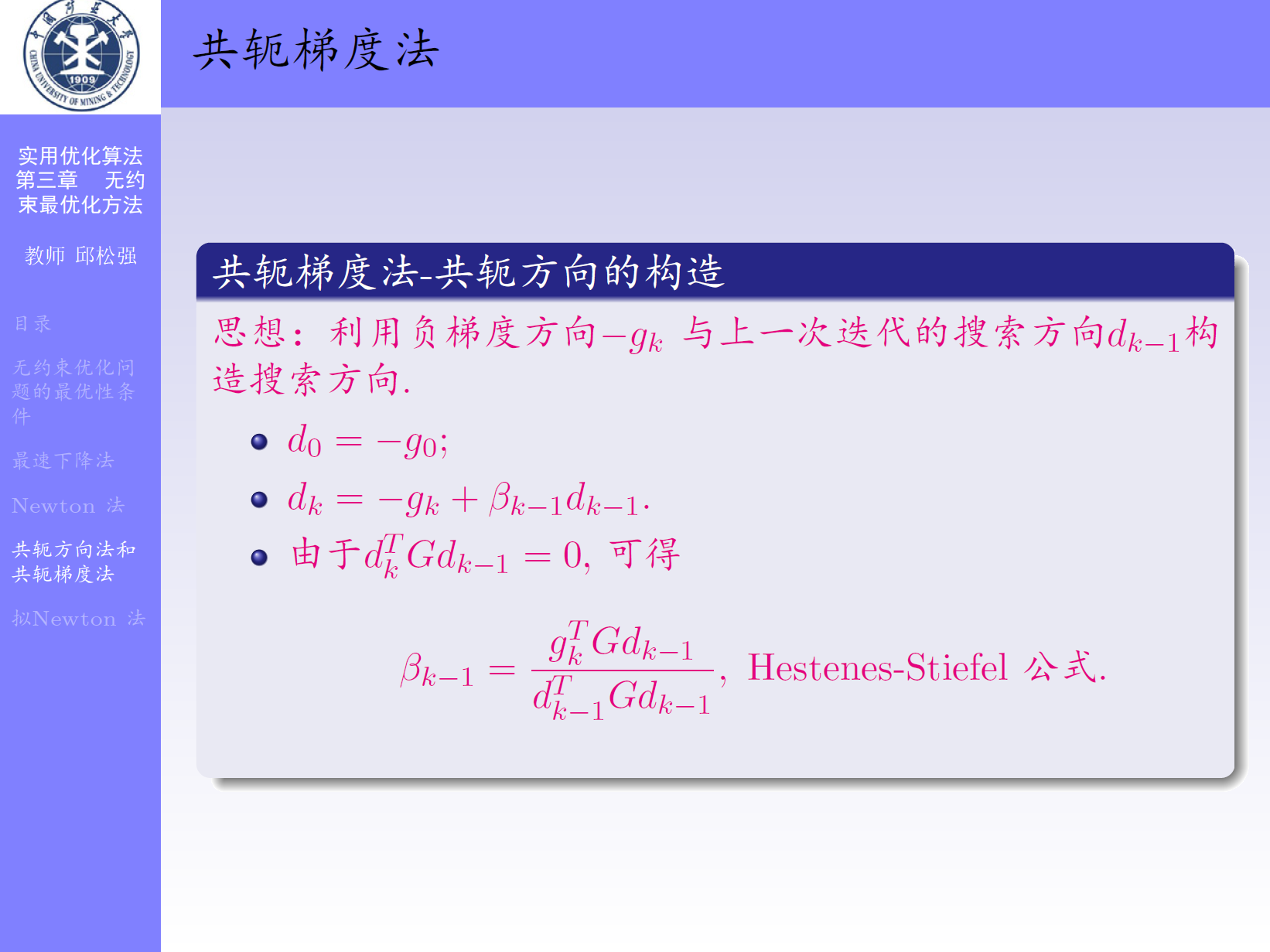hylee老师讲的：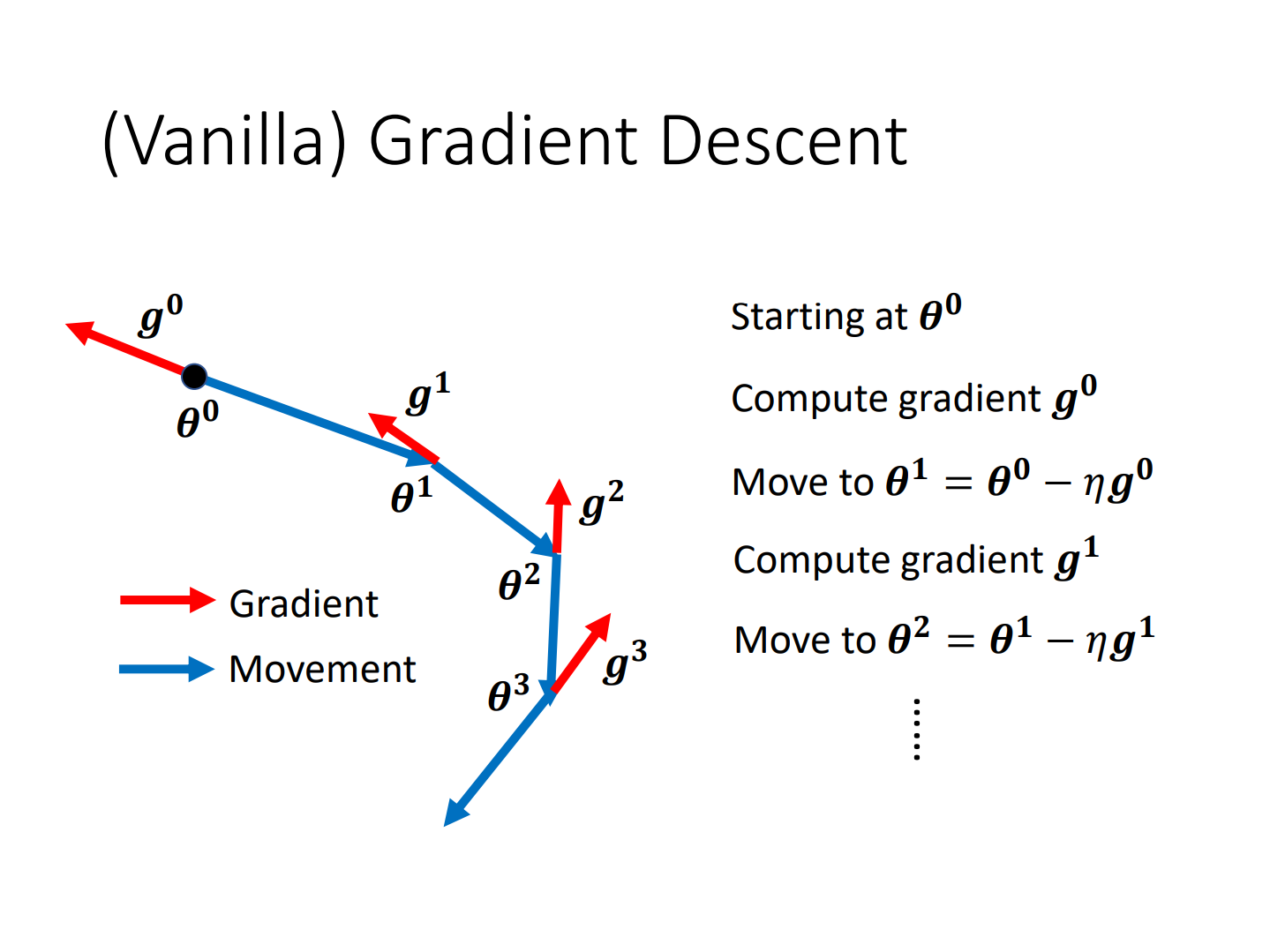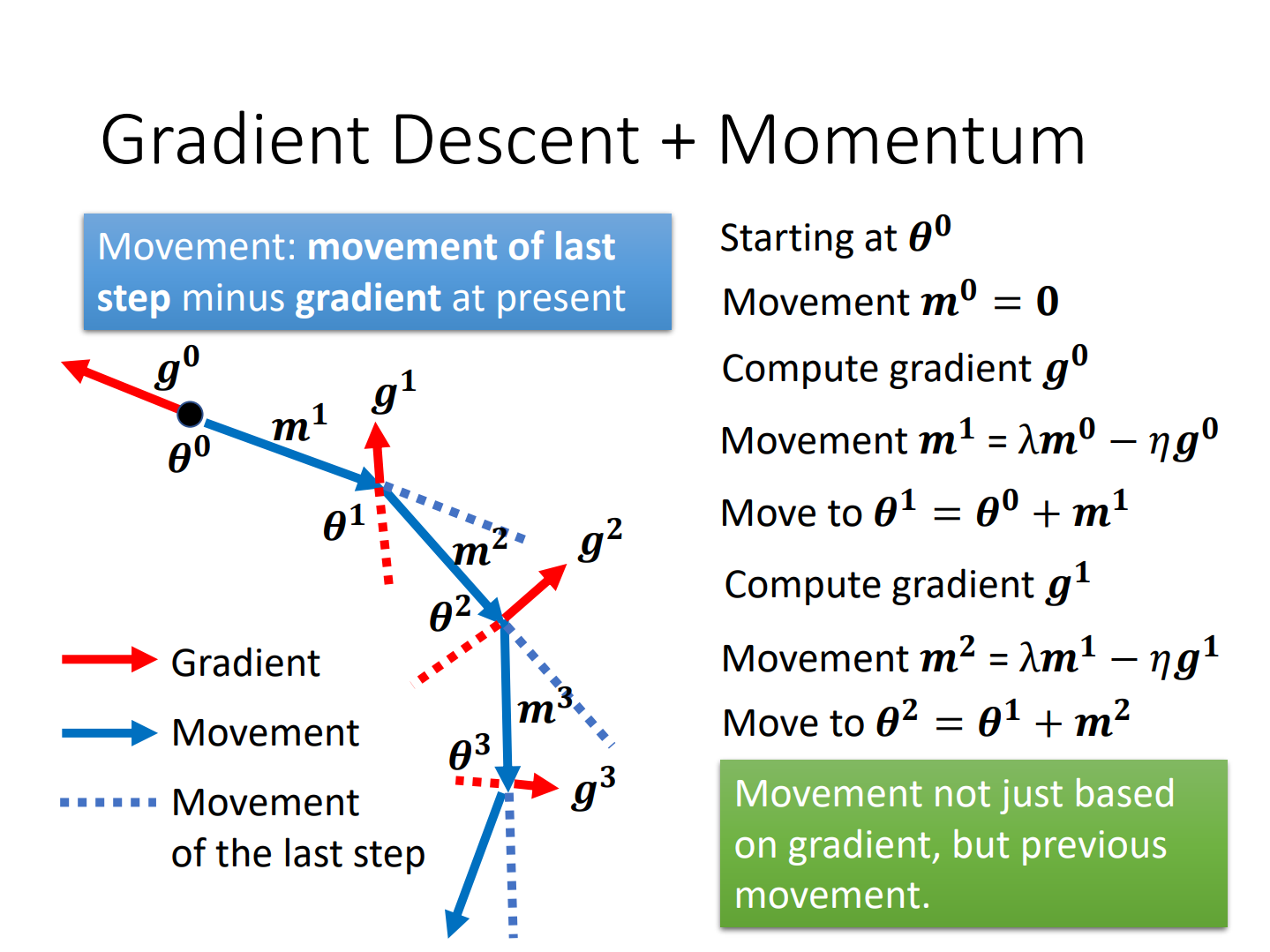### Define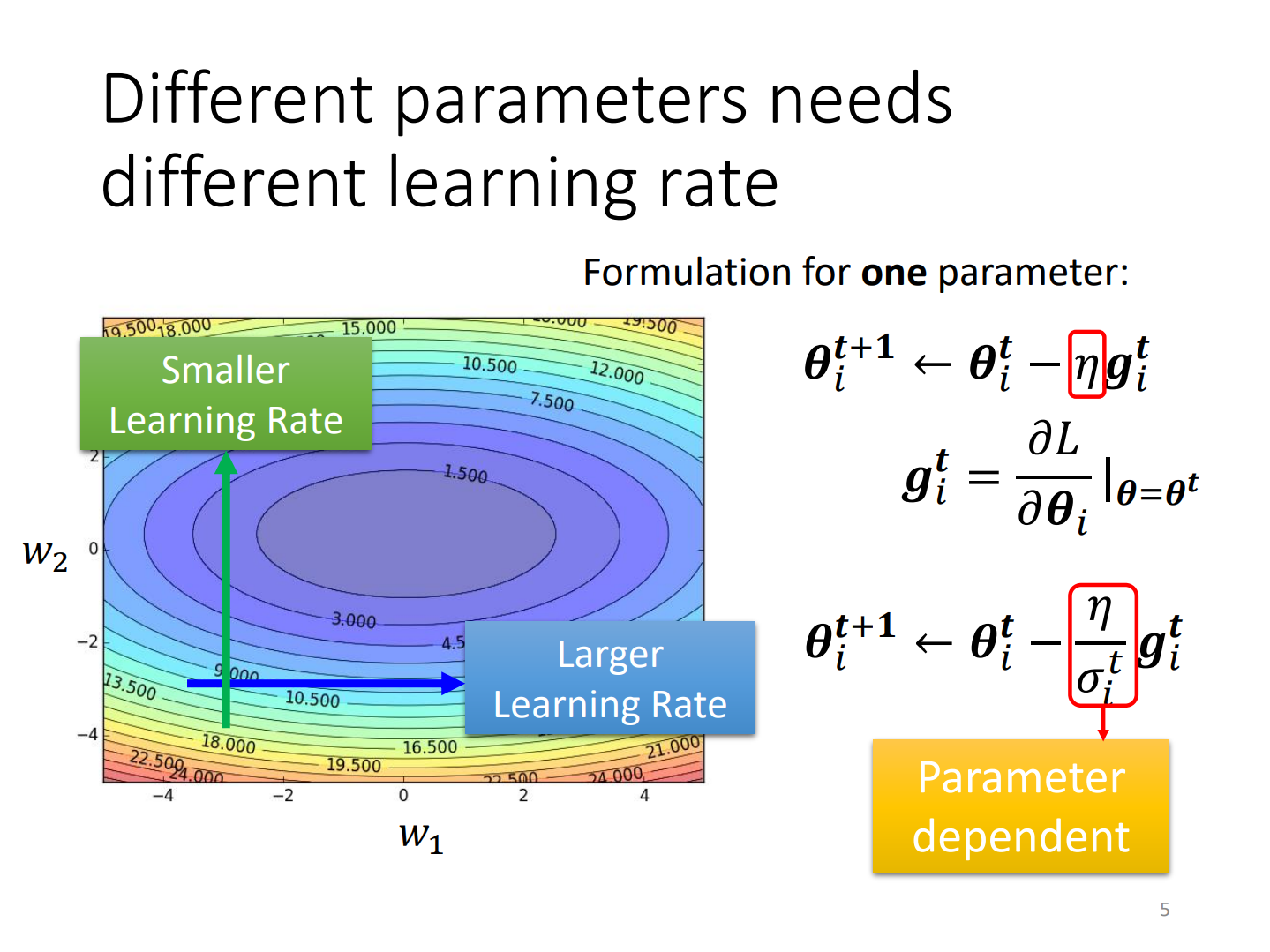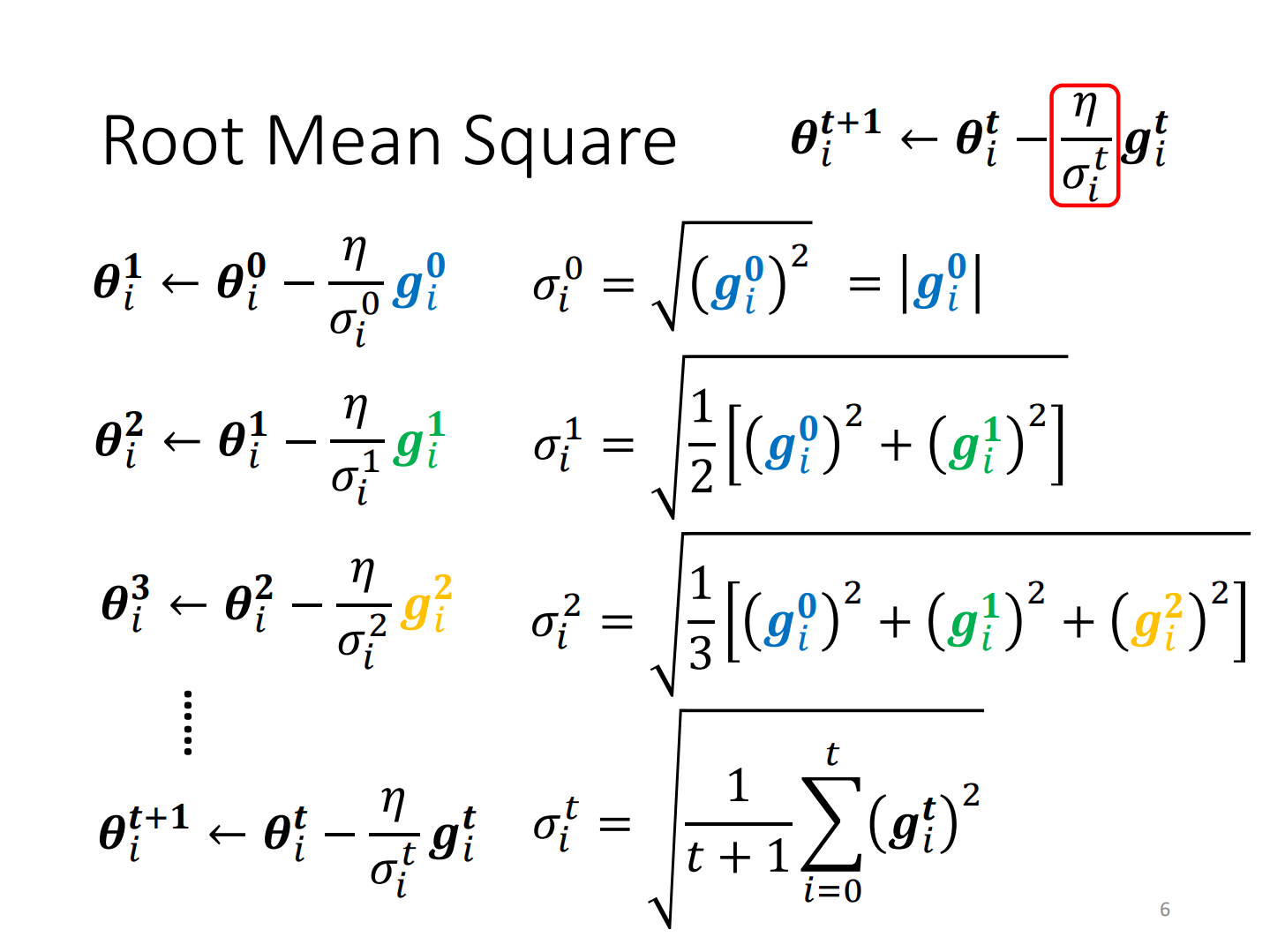root mean square：均方根

### Scheduling

#### Decay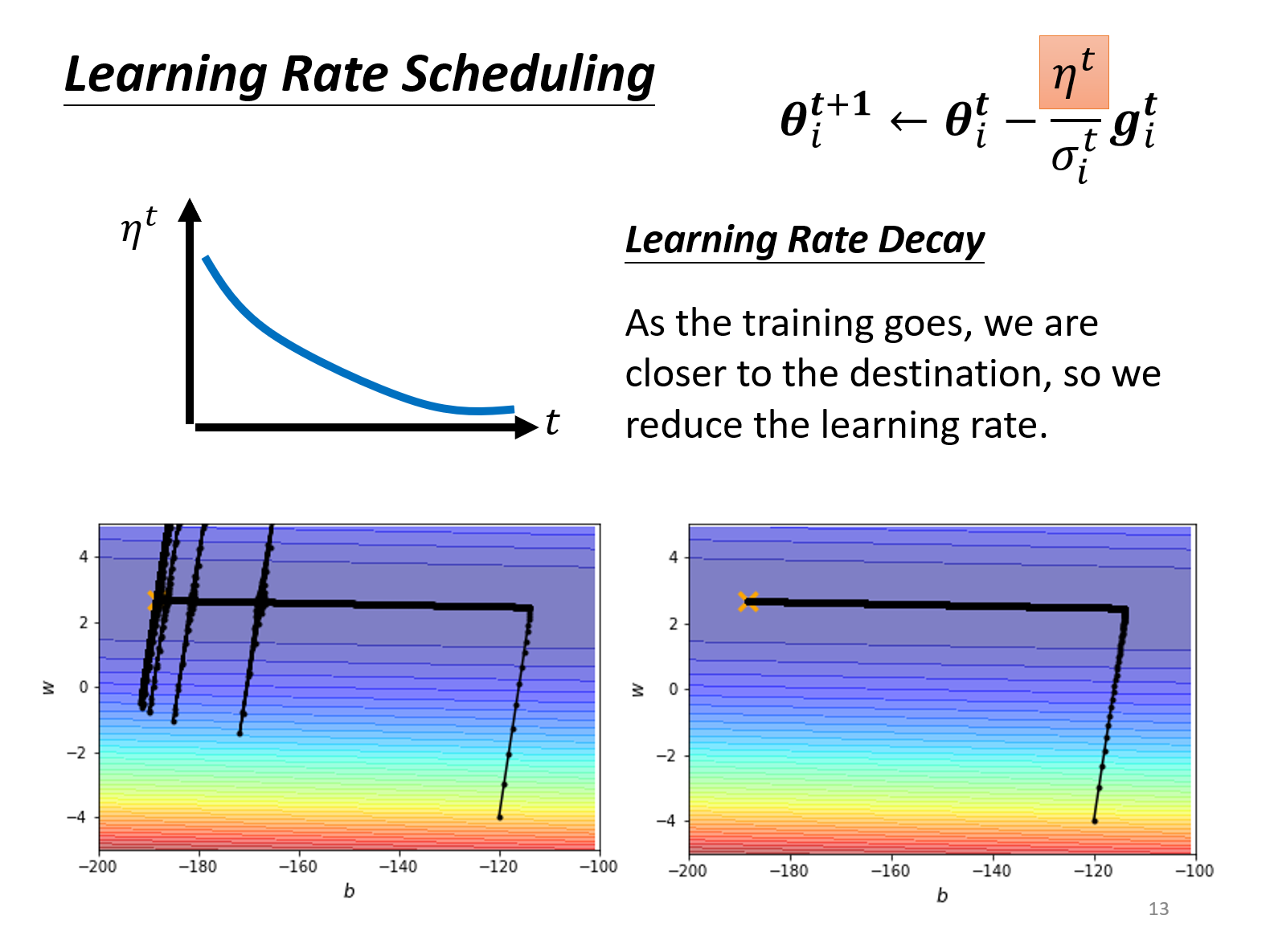#### warmup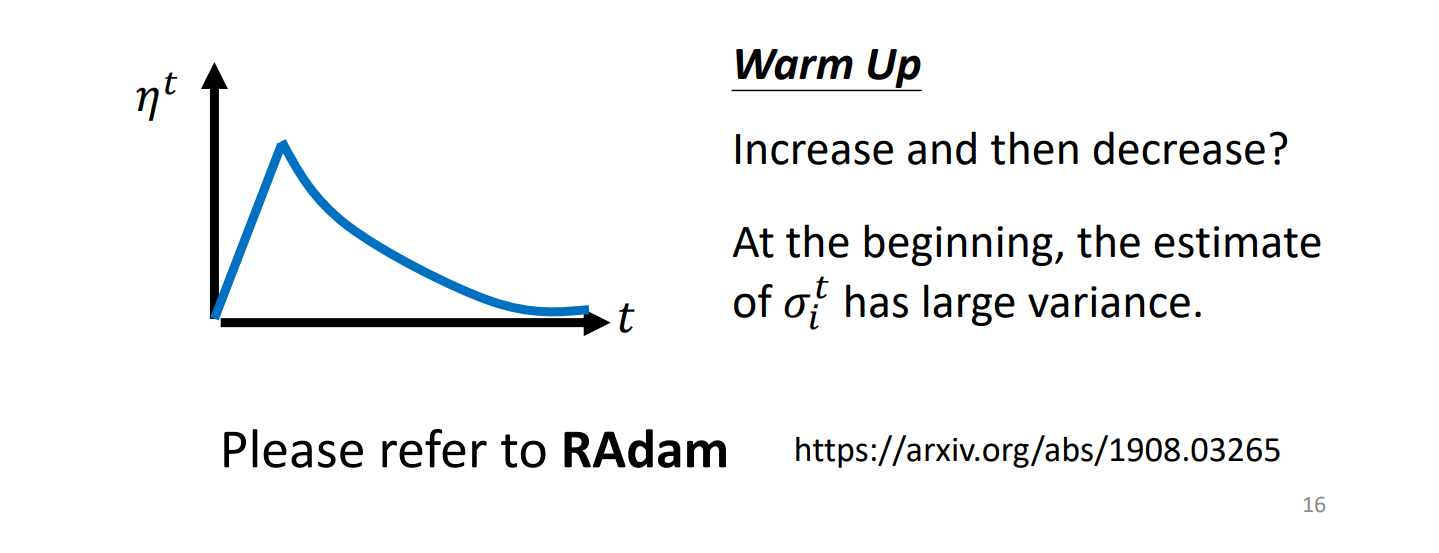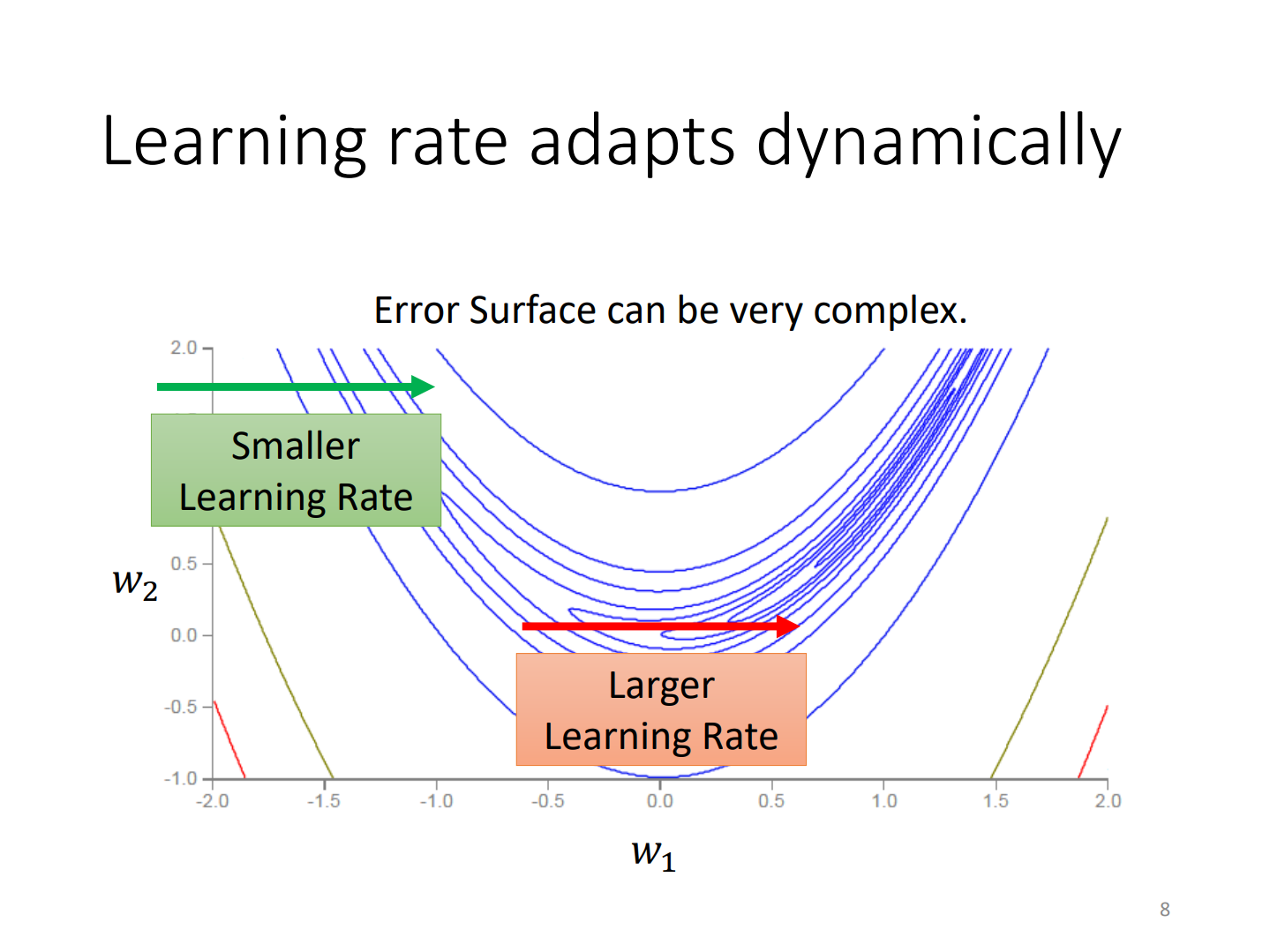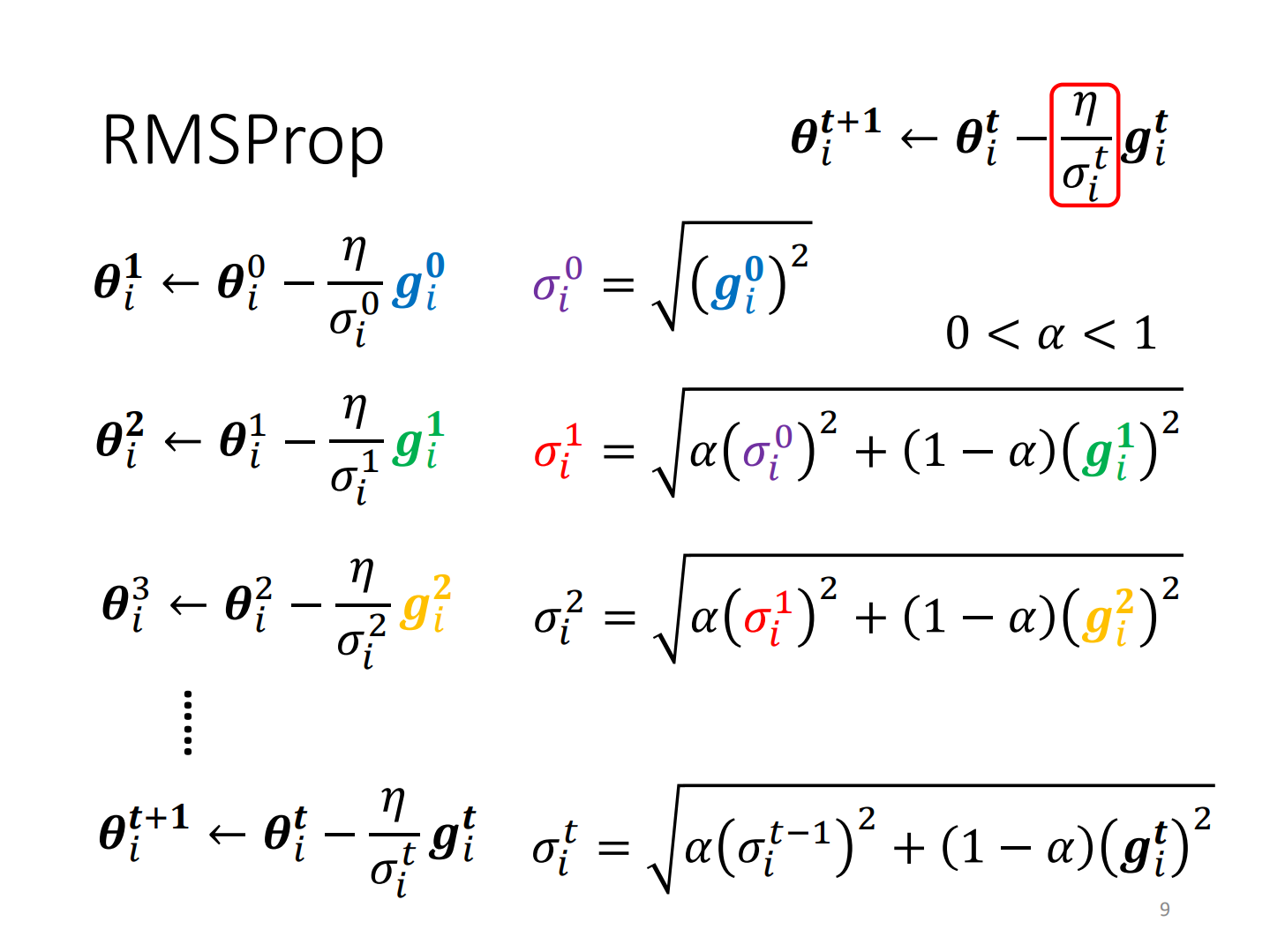RMSProp：RMS+支点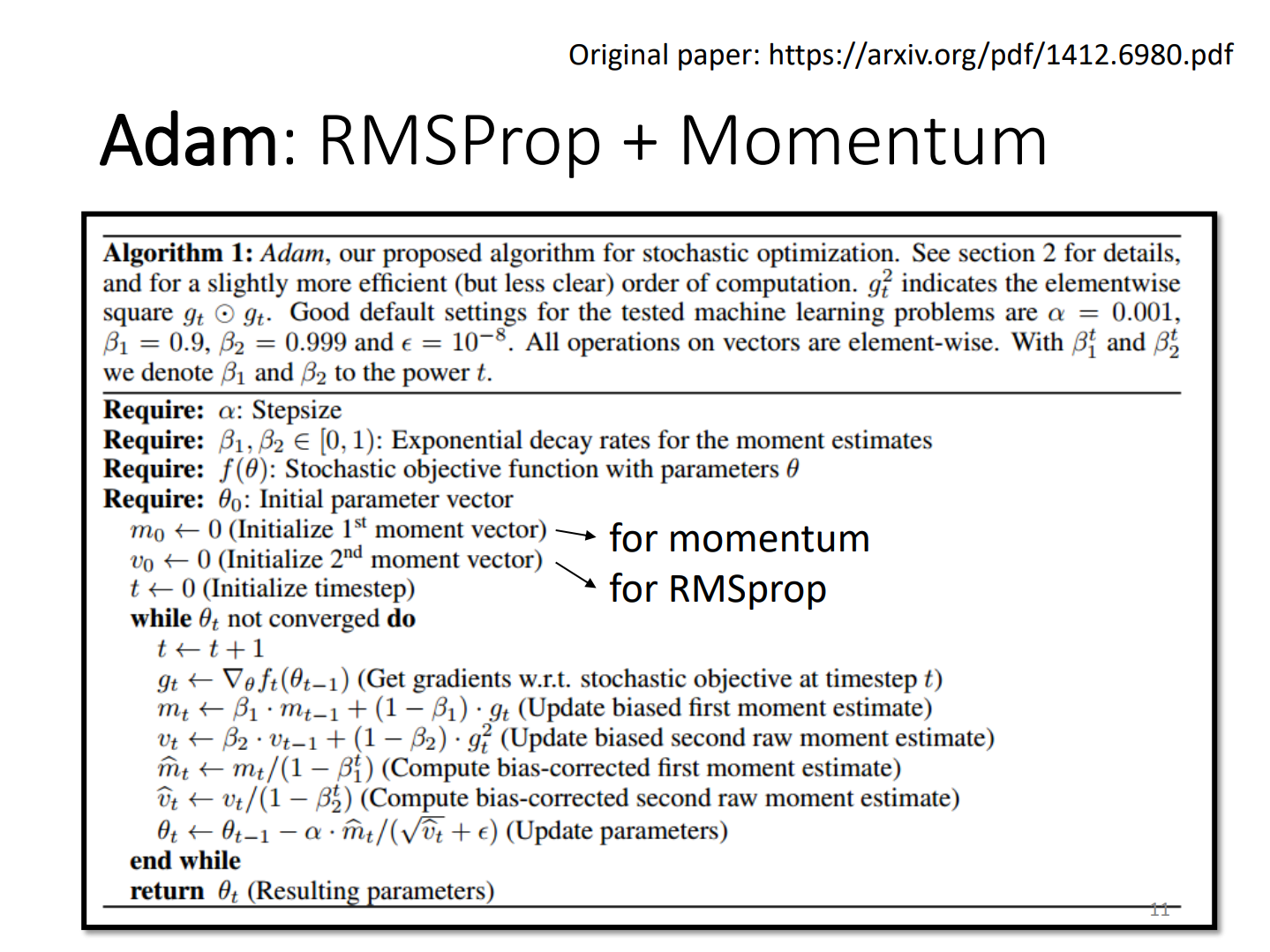## Cross-entropy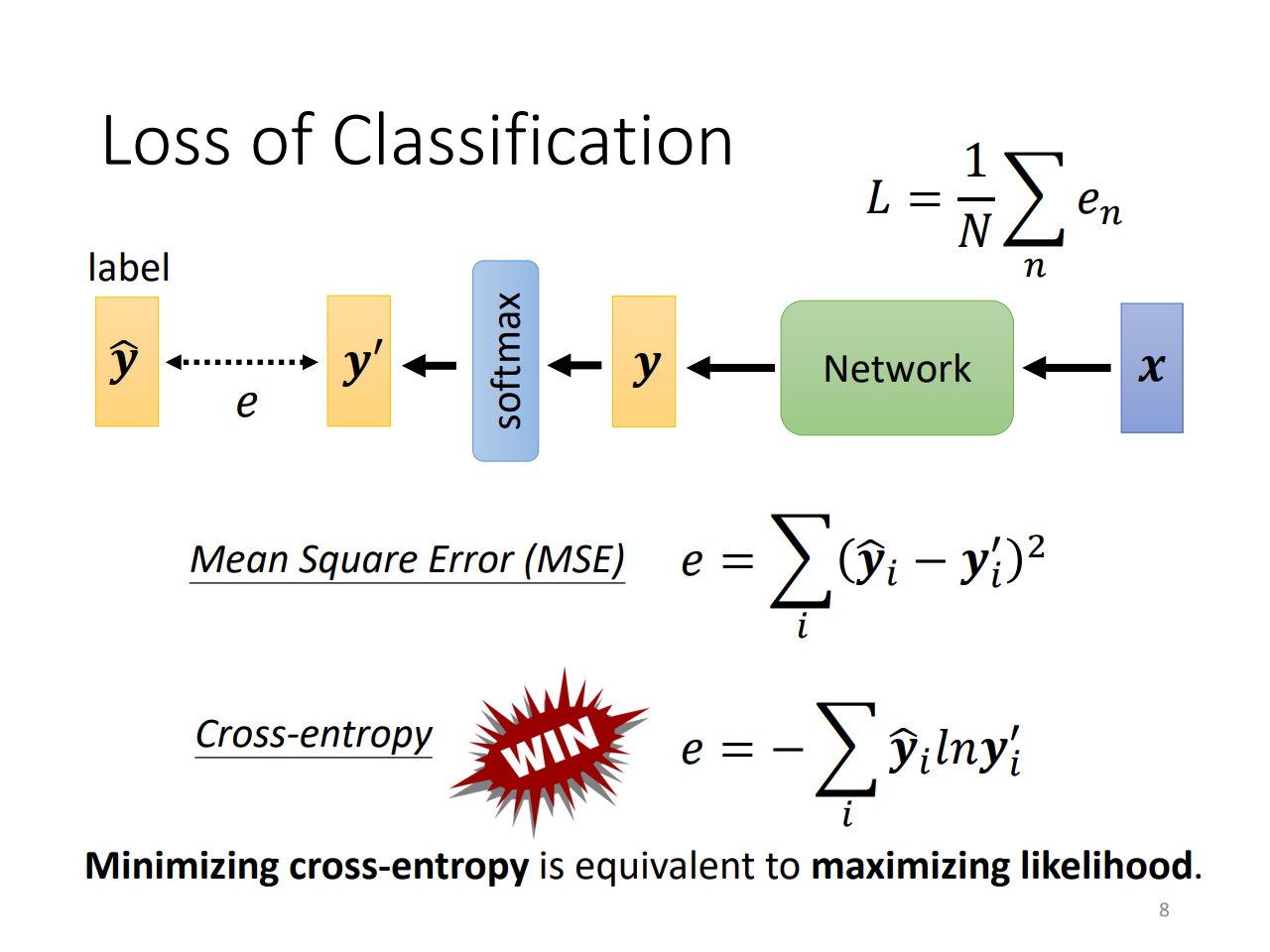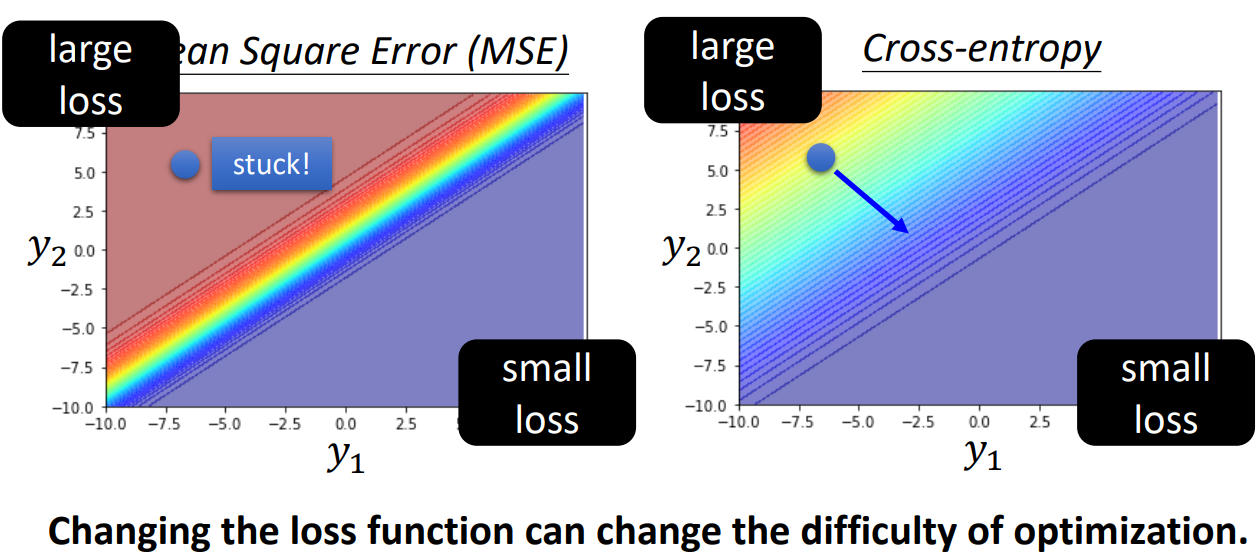loss function会改变optimization的难度！

## Batch Normalization(BN)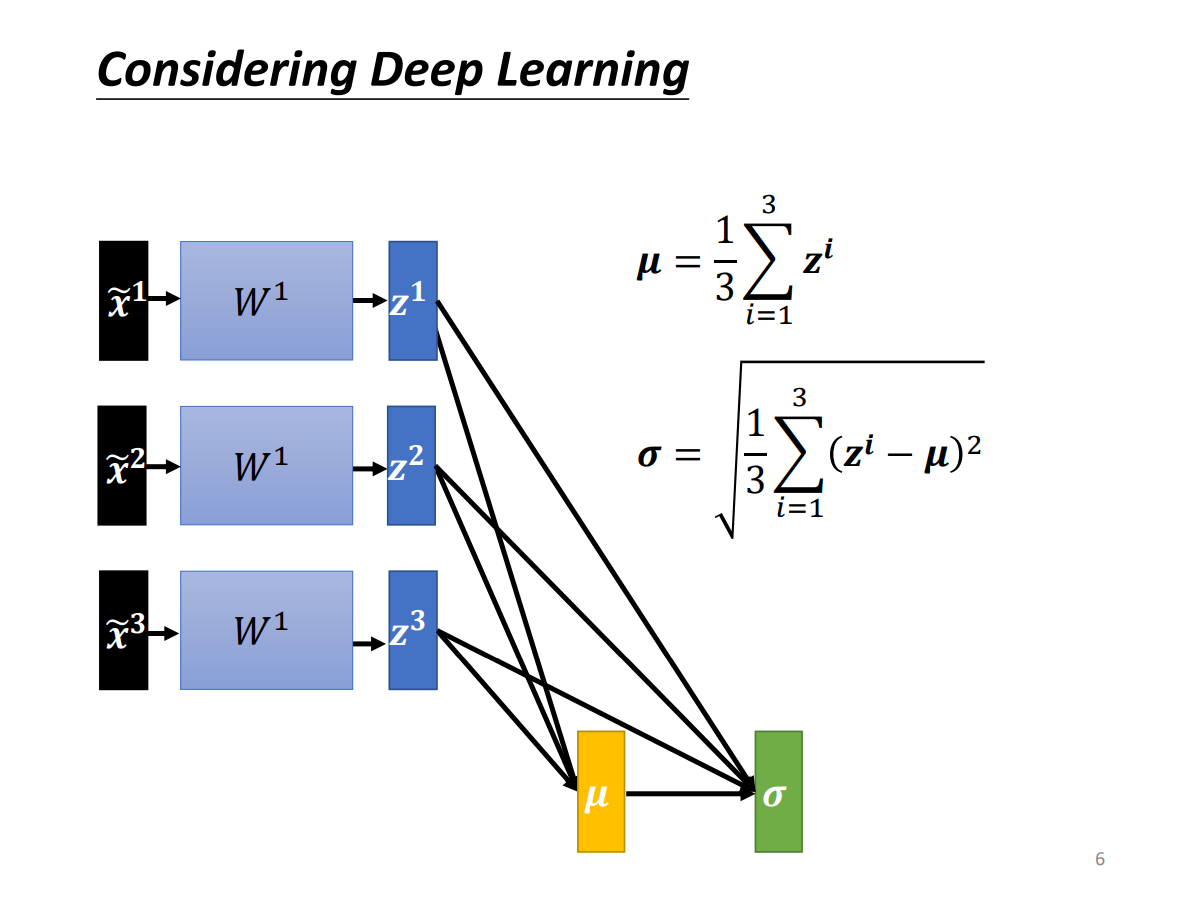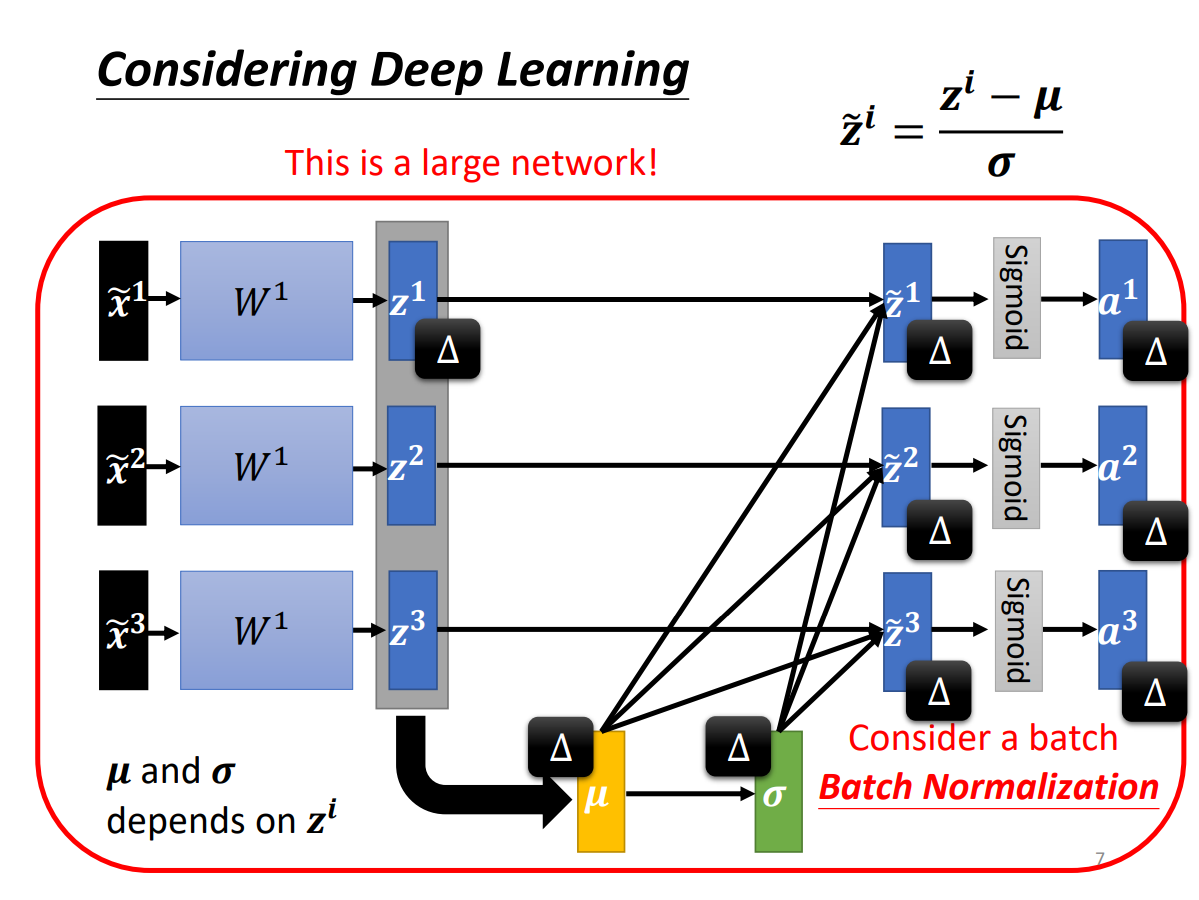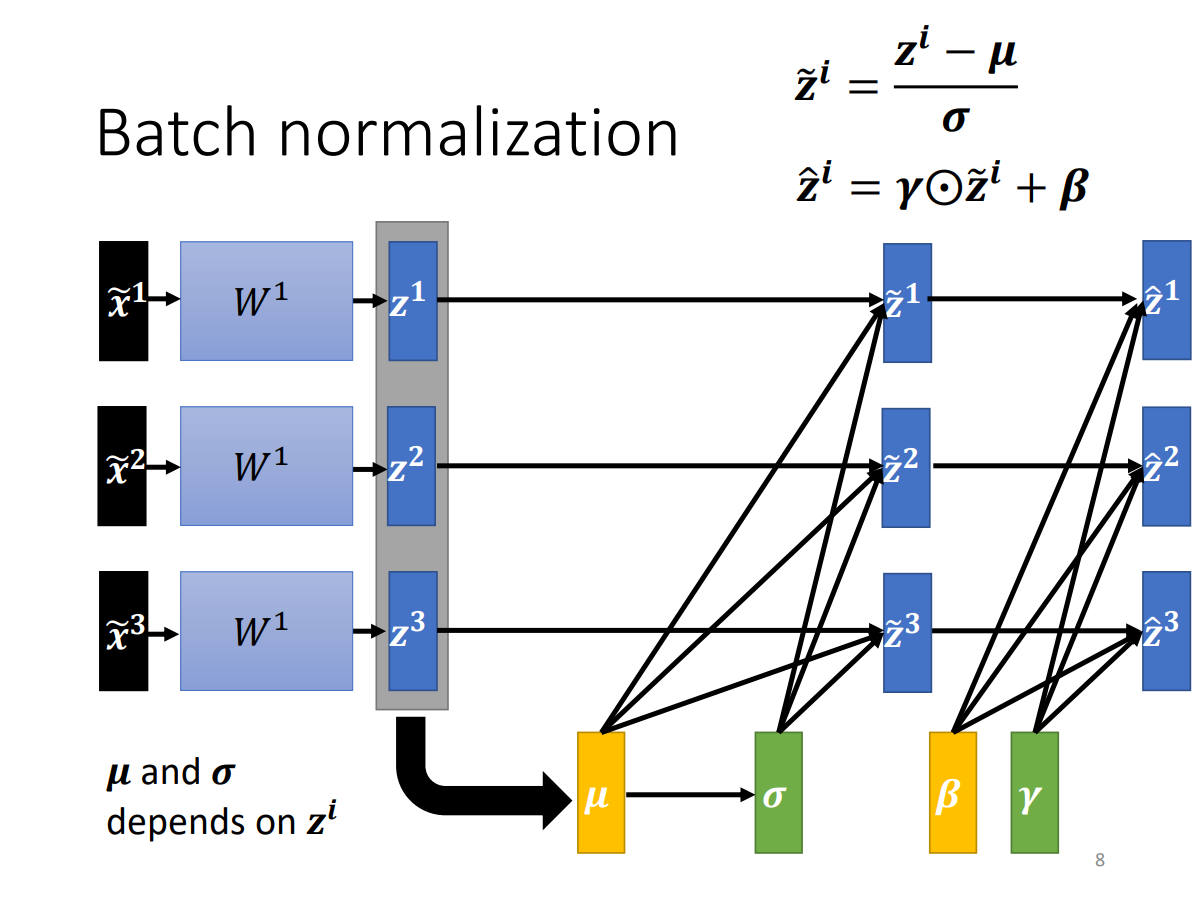# notation

$\hat{y}$：y hat

$e^x$：exponential x

$\sum$：Summation

$y{\prime}$：y prime

logit：softmax的输入

$\tilde{x}$：x tilde, normalization后的x

element-wise product：元素对应乘积

inference：test